### Obliquity (1 of 5)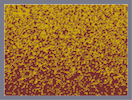Hover over the thumbnail for a full-size version.

Author bobshike author:bobshike bitesized n-art rated 2006-03-16 2006-03-16 4 by 19 people. \$Obliquity (1 of 5)#bobshike#none#00000000000000000000000000000000000000000000000000000000000000000000000000000000000000000000000000000000000000000000000000000000000000000000000000000000000000000000000000000000000000000000000000000000000000000000000000000000000000000000000000000000000000000000000000000000000000000000000000000000000000000000000000000000000000000000000000000000000000000000000000000000000000000000000000000000000000000000000000000000000000000000000000000000000000000000000000000000000000000000000000000000000000000000000000000000000000000000000000000000000000000000000000000000000000000000000000000000000000000000000000000000000000000000000000000000000000000000000000000000000000000000000000000000000000000000000000000000000000000|0^30,570!12^30,564!12^30,558!12^30,552!12^30,546!12^30,540!0^30,534!12^30,528!12^30,522!12^30,516!12^30,510!0^30,504!12^30,498!12^30,492!12^30,486!12^30,480!12^30,474!12^30,468!12^30,462!12^30,456!0^30,450!12^30,444!12^30,438!12^30,432!12^30,426!0^30,420!12^30,414!12^30,408!0^30,402!0^30,396!12^30,390!0^30,384!12^30,378!0^30,372!12^30,366!0^30,360!12^30,354!12^30,348!12^30,342!12^30,336!12^30,330!0^30,324!12^30,318!0^30,312!12^30,306!0^30,300!12^30,294!0^30,288!0^30,282!12^30,276!0^30,270!12^30,264!12^30,258!0^30,252!12^30,246!12^30,240!0^30,234!0^30,228!12^30,222!0^30,216!12^30,210!0^30,204!12^30,198!0^30,192!0^30,186!0^30,180!0^30,174!12^30,168!0^30,162!12^30,156!0^30,150!0^30,144!0^30,138!12^30,132!12^30,126!12^30,120!0^30,114!0^30,108!0^30,102!0^30,96!0^30,90!12^30,84!0^30,78!0^30,72!12^30,66!12^30,60!0^30,54!12^30,48!0^30,42!0^30,36!0^30,30!12^36,570!12^36,564!12^36,558!12^36,552!12^36,546!12^36,540!12^36,534!12^36,528!12^36,522!12^36,516!12^36,510!12^36,504!0^36,498!12^36,492!12^36,486!12^36,480!12^36,474!0^36,468!0^36,462!12^36,456!12^36,450!12^36,444!12^36,438!0^36,432!12^36,426!0^36,420!0^36,414!12^36,408!0^36,402!0^36,396!12^36,390!12^36,384!0^36,378!12^36,372!12^36,366!12^36,360!12^36,354!12^36,348!12^36,342!12^36,336!12^36,330!12^36,324!0^36,318!12^36,312!12^36,306!0^36,300!12^36,294!0^36,288!12^36,282!0^36,276!12^36,270!12^36,264!0^36,258!12^36,252!12^36,246!12^36,240!0^36,234!0^36,228!12^36,222!12^36,216!0^36,210!12^36,204!0^36,198!0^36,192!0^36,186!12^36,180!12^36,174!0^36,168!0^36,162!0^36,156!0^36,150!0^36,144!0^36,138!0^36,132!0^36,126!0^36,120!0^36,114!12^36,108!0^36,102!0^36,96!0^36,90!0^36,84!0^36,78!12^36,72!12^36,66!0^36,60!0^36,54!12^36,48!0^36,42!0^36,36!0^36,30!12^42,570!12^42,564!0^42,558!12^42,552!12^42,546!12^42,540!12^42,534!12^42,528!12^42,522!12^42,516!12^42,510!12^42,504!12^42,498!12^42,492!0^42,486!12^42,480!12^42,474!0^42,468!12^42,462!12^42,456!12^42,450!12^42,444!12^42,438!12^42,432!0^42,426!12^42,420!12^42,414!12^42,408!12^42,402!0^42,396!0^42,390!12^42,384!12^42,378!12^42,372!12^42,366!12^42,360!0^42,354!12^42,348!12^42,342!12^42,336!12^42,330!0^42,324!0^42,318!12^42,312!12^42,306!0^42,300!12^42,294!12^42,288!12^42,282!12^42,276!0^42,270!0^42,264!12^42,258!0^42,252!0^42,246!12^42,240!0^42,234!12^42,228!0^42,222!0^42,216!12^42,210!12^42,204!12^42,198!12^42,192!0^42,186!0^42,180!12^42,174!12^42,168!0^42,162!12^42,156!0^42,150!12^42,144!0^42,138!12^42,132!0^42,126!12^42,120!0^42,114!0^42,108!12^42,102!12^42,96!12^42,90!12^42,84!0^42,78!0^42,72!0^42,66!0^42,60!0^42,54!0^42,48!12^42,42!12^42,36!0^42,30!12^48,570!12^48,564!12^48,558!12^48,552!12^48,546!12^48,540!12^48,534!12^48,528!12^48,522!12^48,516!12^48,510!12^48,504!12^48,498!12^48,492!12^48,486!12^48,480!12^48,474!12^48,468!12^48,462!0^48,456!12^48,450!12^48,444!0^48,438!12^48,432!0^48,426!12^48,420!0^48,414!12^48,408!0^48,402!0^48,396!12^48,390!12^48,384!12^48,378!12^48,372!12^48,366!0^48,360!0^48,354!12^48,348!12^48,342!0^48,336!12^48,330!12^48,324!12^48,318!0^48,312!0^48,306!0^48,300!12^48,294!12^48,288!12^48,282!12^48,276!12^48,270!12^48,264!0^48,258!12^48,252!12^48,246!0^48,240!12^48,234!12^48,228!0^48,222!12^48,216!0^48,210!0^48,204!0^48,198!12^48,192!12^48,186!12^48,180!0^48,174!12^48,168!12^48,162!12^48,156!0^48,150!12^48,144!0^48,138!0^48,132!12^48,126!12^48,120!12^48,114!0^48,108!12^48,102!0^48,96!0^48,90!0^48,84!0^48,78!0^48,72!0^48,66!0^48,60!0^48,54!0^48,48!0^48,42!0^48,36!0^48,30!12^54,570!12^54,564!0^54,558!12^54,552!12^54,546!12^54,540!0^54,534!12^54,528!12^54,522!12^54,516!12^54,510!12^54,504!0^54,498!12^54,492!12^54,486!12^54,480!0^54,474!12^54,468!12^54,462!12^54,456!0^54,450!0^54,444!0^54,438!12^54,432!0^54,426!0^54,420!12^54,414!0^54,408!12^54,402!0^54,396!0^54,390!12^54,384!12^54,378!0^54,372!0^54,366!12^54,360!12^54,354!0^54,348!12^54,342!0^54,336!12^54,330!12^54,324!12^54,318!0^54,312!0^54,306!0^54,300!0^54,294!0^54,288!12^54,282!12^54,276!0^54,270!0^54,264!12^54,258!12^54,252!0^54,246!12^54,240!12^54,234!12^54,228!12^54,222!0^54,216!12^54,210!12^54,204!12^54,198!0^54,192!12^54,186!0^54,180!12^54,174!12^54,168!0^54,162!0^54,156!0^54,150!0^54,144!12^54,138!0^54,132!0^54,126!0^54,120!12^54,114!0^54,108!0^54,102!0^54,96!12^54,90!12^54,84!0^54,78!0^54,72!12^54,66!0^54,60!0^54,54!12^54,48!0^54,42!0^54,36!0^54,30!12^60,570!12^60,564!12^60,558!12^60,552!12^60,546!12^60,540!12^60,534!12^60,528!0^60,522!12^60,516!12^60,510!12^60,504!12^60,498!0^60,492!12^60,486!12^60,480!12^60,474!0^60,468!0^60,462!12^60,456!12^60,450!12^60,444!12^60,438!0^60,432!0^60,426!0^60,420!12^60,414!0^60,408!0^60,402!12^60,396!12^60,390!12^60,384!12^60,378!12^60,372!0^60,366!12^60,360!12^60,354!12^60,348!12^60,342!12^60,336!12^60,330!12^60,324!12^60,318!12^60,312!12^60,306!12^60,300!12^60,294!12^60,288!0^60,282!12^60,276!12^60,270!12^60,264!12^60,258!12^60,252!12^60,246!12^60,240!12^60,234!0^60,228!0^60,222!0^60,216!12^60,210!12^60,204!12^60,198!0^60,192!0^60,186!12^60,180!12^60,174!12^60,168!0^60,162!0^60,156!12^60,150!0^60,144!12^60,138!12^60,132!12^60,126!0^60,120!0^60,114!0^60,108!0^60,102!0^60,96!12^60,90!0^60,84!12^60,78!12^60,72!0^60,66!12^60,60!0^60,54!0^60,48!12^60,42!0^60,36!0^60,30!12^66,570!12^66,564!0^66,558!12^66,552!12^66,546!12^66,540!12^66,534!12^66,528!12^66,522!0^66,516!12^66,510!12^66,504!12^66,498!12^66,492!12^66,486!0^66,480!0^66,474!12^66,468!12^66,462!0^66,456!0^66,450!12^66,444!12^66,438!0^66,432!12^66,426!12^66,420!12^66,414!12^66,408!12^66,402!0^66,396!0^66,390!12^66,384!0^66,378!12^66,372!12^66,366!12^66,360!12^66,354!0^66,348!12^66,342!12^66,336!0^66,330!0^66,324!12^66,318!12^66,312!0^66,306!12^66,300!0^66,294!0^66,288!12^66,282!0^66,276!0^66,270!12^66,264!12^66,258!0^66,252!0^66,246!12^66,240!12^66,234!0^66,228!12^66,222!0^66,216!12^66,210!12^66,204!12^66,198!12^66,192!0^66,186!12^66,180!12^66,174!12^66,168!12^66,162!0^66,156!0^66,150!0^66,144!0^66,138!0^66,132!0^66,126!0^66,120!0^66,114!12^66,108!0^66,102!0^66,96!12^66,90!0^66,84!0^66,78!0^66,72!0^66,66!12^66,60!12^66,54!0^66,48!12^66,42!12^66,36!12^66,30!12^72,570!12^72,564!12^72,558!12^72,552!12^72,546!12^72,540!12^72,534!12^72,528!12^72,522!12^72,516!12^72,510!12^72,504!12^72,498!0^72,492!12^72,486!12^72,480!12^72,474!12^72,468!12^72,462!12^72,456!12^72,450!12^72,444!12^72,438!12^72,432!12^72,426!12^72,420!12^72,414!0^72,408!0^72,402!12^72,396!12^72,390!0^72,384!12^72,378!12^72,372!0^72,366!0^72,360!12^72,354!0^72,348!12^72,342!12^72,336!12^72,330!0^72,324!0^72,318!12^72,312!0^72,306!12^72,300!12^72,294!12^72,288!12^72,282!12^72,276!12^72,270!12^72,264!0^72,258!0^72,252!12^72,246!12^72,240!0^72,234!12^72,228!0^72,222!12^72,216!0^72,210!0^72,204!0^72,198!12^72,192!0^72,186!12^72,180!0^72,174!0^72,168!12^72,162!0^72,156!0^72,150!12^72,144!12^72,138!12^72,132!0^72,126!0^72,120!0^72,114!12^72,108!12^72,102!12^72,96!0^72,90!12^72,84!12^72,78!0^72,72!0^72,66!12^72,60!0^72,54!12^72,48!12^72,42!0^72,36!0^72,30!12^78,570!0^78,564!12^78,558!12^78,552!0^78,546!12^78,540!12^78,534!12^78,528!12^78,522!12^78,516!12^78,510!12^78,504!12^78,498!12^78,492!12^78,486!12^78,480!12^78,474!12^78,468!12^78,462!12^78,456!0^78,450!12^78,444!12^78,438!12^78,432!0^78,426!12^78,420!12^78,414!12^78,408!12^78,402!12^78,396!12^78,390!0^78,384!0^78,378!12^78,372!12^78,366!12^78,360!12^78,354!0^78,348!0^78,342!12^78,336!12^78,330!12^78,324!12^78,318!12^78,312!0^78,306!12^78,300!0^78,294!12^78,288!0^78,282!0^78,276!0^78,270!12^78,264!0^78,258!0^78,252!12^78,246!12^78,240!0^78,234!12^78,228!12^78,222!12^78,216!0^78,210!12^78,204!12^78,198!12^78,192!12^78,186!0^78,180!12^78,174!0^78,168!12^78,162!0^78,156!0^78,150!12^78,144!12^78,138!0^78,132!12^78,126!12^78,120!12^78,114!0^78,108!0^78,102!0^78,96!0^78,90!0^78,84!0^78,78!0^78,72!12^78,66!0^78,60!0^78,54!0^78,48!12^78,42!0^78,36!0^78,30!12^84,570!12^84,564!0^84,558!12^84,552!12^84,546!12^84,540!0^84,534!12^84,528!12^84,522!12^84,516!12^84,510!12^84,504!0^84,498!12^84,492!12^84,486!12^84,480!12^84,474!0^84,468!12^84,462!12^84,456!0^84,450!12^84,444!0^84,438!0^84,432!12^84,426!0^84,420!12^84,414!12^84,408!12^84,402!12^84,396!0^84,390!12^84,384!12^84,378!0^84,372!12^84,366!12^84,360!12^84,354!0^84,348!0^84,342!0^84,336!12^84,330!0^84,324!12^84,318!0^84,312!12^84,306!12^84,300!12^84,294!0^84,288!0^84,282!0^84,276!12^84,270!12^84,264!12^84,258!12^84,252!12^84,246!0^84,240!12^84,234!12^84,228!12^84,222!12^84,216!0^84,210!12^84,204!12^84,198!0^84,192!0^84,186!0^84,180!0^84,174!0^84,168!0^84,162!0^84,156!12^84,150!12^84,144!12^84,138!12^84,132!0^84,126!0^84,120!12^84,114!12^84,108!12^84,102!0^84,96!12^84,90!0^84,84!0^84,78!0^84,72!12^84,66!0^84,60!12^84,54!12^84,48!0^84,42!0^84,36!0^84,30!12^90,570!0^90,564!12^90,558!12^90,552!12^90,546!12^90,540!12^90,534!0^90,528!12^90,522!0^90,516!12^90,510!12^90,504!0^90,498!12^90,492!12^90,486!12^90,480!12^90,474!12^90,468!12^90,462!12^90,456!12^90,450!12^90,444!0^90,438!12^90,432!12^90,426!12^90,420!0^90,414!12^90,408!12^90,402!0^90,396!12^90,390!0^90,384!12^90,378!0^90,372!0^90,366!12^90,360!0^90,354!12^90,348!12^90,342!12^90,336!12^90,330!0^90,324!12^90,318!12^90,312!0^90,306!0^90,300!12^90,294!0^90,288!0^90,282!0^90,276!12^90,270!12^90,264!12^90,258!0^90,252!12^90,246!0^90,240!0^90,234!0^90,228!0^90,222!12^90,216!12^90,210!12^90,204!0^90,198!12^90,192!12^90,186!0^90,180!0^90,174!0^90,168!12^90,162!12^90,156!12^90,150!0^90,144!12^90,138!12^90,132!0^90,126!0^90,120!12^90,114!0^90,108!0^90,102!12^90,96!0^90,90!0^90,84!0^90,78!0^90,72!12^90,66!0^90,60!0^90,54!0^90,48!0^90,42!0^90,36!0^90,30!12^96,570!12^96,564!12^96,558!12^96,552!12^96,546!12^96,540!12^96,534!12^96,528!0^96,522!12^96,516!12^96,510!12^96,504!12^96,498!0^96,492!12^96,486!12^96,480!12^96,474!12^96,468!0^96,462!12^96,456!0^96,450!12^96,444!12^96,438!12^96,432!12^96,426!12^96,420!0^96,414!0^96,408!0^96,402!12^96,396!0^96,390!12^96,384!12^96,378!12^96,372!12^96,366!0^96,360!12^96,354!12^96,348!0^96,342!0^96,336!0^96,330!12^96,324!12^96,318!12^96,312!12^96,306!12^96,300!12^96,294!0^96,288!0^96,282!12^96,276!12^96,270!0^96,264!0^96,258!0^96,252!12^96,246!0^96,240!12^96,234!12^96,228!0^96,222!12^96,216!12^96,210!0^96,204!0^96,198!0^96,192!12^96,186!12^96,180!0^96,174!0^96,168!12^96,162!0^96,156!0^96,150!0^96,144!0^96,138!12^96,132!12^96,126!12^96,120!0^96,114!0^96,108!12^96,102!0^96,96!0^96,90!0^96,84!0^96,78!0^96,72!12^96,66!0^96,60!12^96,54!12^96,48!12^96,42!12^96,36!12^96,30!12^102,570!12^102,564!12^102,558!12^102,552!12^102,546!12^102,540!12^102,534!12^102,528!12^102,522!12^102,516!12^102,510!0^102,504!12^102,498!12^102,492!12^102,486!12^102,480!12^102,474!12^102,468!12^102,462!12^102,456!12^102,450!12^102,444!12^102,438!12^102,432!12^102,426!12^102,420!12^102,414!0^102,408!12^102,402!0^102,396!12^102,390!12^102,384!12^102,378!0^102,372!0^102,366!12^102,360!0^102,354!12^102,348!12^102,342!12^102,336!0^102,330!12^102,324!0^102,318!0^102,312!12^102,306!0^102,300!0^102,294!12^102,288!12^102,282!12^102,276!0^102,270!0^102,264!0^102,258!12^102,252!12^102,246!12^102,240!0^102,234!12^102,228!0^102,222!0^102,216!12^102,210!0^102,204!12^102,198!12^102,192!0^102,186!0^102,180!12^102,174!12^102,168!12^102,162!12^102,156!0^102,150!12^102,144!0^102,138!0^102,132!0^102,126!0^102,120!0^102,114!0^102,108!12^102,102!0^102,96!12^102,90!0^102,84!0^102,78!12^102,72!12^102,66!0^102,60!12^102,54!12^102,48!12^102,42!12^102,36!0^102,30!12^108,570!12^108,564!12^108,558!12^108,552!12^108,546!0^108,540!12^108,534!12^108,528!12^108,522!12^108,516!12^108,510!12^108,504!12^108,498!12^108,492!12^108,486!0^108,480!12^108,474!12^108,468!12^108,462!12^108,456!12^108,450!12^108,444!0^108,438!12^108,432!12^108,426!12^108,420!12^108,414!12^108,408!12^108,402!12^108,396!12^108,390!12^108,384!12^108,378!12^108,372!0^108,366!12^108,360!12^108,354!12^108,348!12^108,342!12^108,336!0^108,330!0^108,324!12^108,318!0^108,312!12^108,306!0^108,300!12^108,294!12^108,288!0^108,282!0^108,276!0^108,270!0^108,264!0^108,258!0^108,252!0^108,246!0^108,240!0^108,234!0^108,228!12^108,222!0^108,216!12^108,210!12^108,204!12^108,198!0^108,192!12^108,186!12^108,180!0^108,174!12^108,168!0^108,162!0^108,156!0^108,150!0^108,144!0^108,138!12^108,132!12^108,126!0^108,120!0^108,114!0^108,108!12^108,102!0^108,96!12^108,90!0^108,84!0^108,78!0^108,72!0^108,66!0^108,60!0^108,54!0^108,48!0^108,42!0^108,36!0^108,30!12^114,570!0^114,564!12^114,558!0^114,552!12^114,546!12^114,540!12^114,534!12^114,528!12^114,522!0^114,516!12^114,510!12^114,504!12^114,498!12^114,492!12^114,486!0^114,480!12^114,474!12^114,468!12^114,462!12^114,456!12^114,450!12^114,444!12^114,438!12^114,432!12^114,426!12^114,420!12^114,414!12^114,408!12^114,402!12^114,396!0^114,390!12^114,384!0^114,378!12^114,372!12^114,366!0^114,360!12^114,354!0^114,348!12^114,342!12^114,336!0^114,330!12^114,324!12^114,318!12^114,312!12^114,306!12^114,300!12^114,294!0^114,288!0^114,282!0^114,276!0^114,270!12^114,264!0^114,258!12^114,252!0^114,246!12^114,240!0^114,234!12^114,228!12^114,222!0^114,216!12^114,210!0^114,204!0^114,198!12^114,192!0^114,186!0^114,180!0^114,174!12^114,168!0^114,162!12^114,156!12^114,150!0^114,144!12^114,138!12^114,132!12^114,126!12^114,120!12^114,114!0^114,108!12^114,102!0^114,96!0^114,90!0^114,84!0^114,78!0^114,72!12^114,66!0^114,60!0^114,54!0^114,48!0^114,42!12^114,36!0^114,30!12^120,570!12^120,564!12^120,558!12^120,552!12^120,546!12^120,540!12^120,534!12^120,528!12^120,522!12^120,516!12^120,510!0^120,504!0^120,498!12^120,492!0^120,486!12^120,480!0^120,474!12^120,468!12^120,462!12^120,456!12^120,450!12^120,444!12^120,438!12^120,432!0^120,426!12^120,420!0^120,414!12^120,408!12^120,402!12^120,396!12^120,390!0^120,384!12^120,378!12^120,372!0^120,366!12^120,360!0^120,354!12^120,348!12^120,342!12^120,336!0^120,330!12^120,324!12^120,318!12^120,312!12^120,306!12^120,300!12^120,294!12^120,288!0^120,282!12^120,276!12^120,270!0^120,264!0^120,258!12^120,252!0^120,246!0^120,240!12^120,234!12^120,228!0^120,222!12^120,216!12^120,210!0^120,204!0^120,198!0^120,192!0^120,186!12^120,180!0^120,174!0^120,168!12^120,162!12^120,156!0^120,150!0^120,144!12^120,138!0^120,132!0^120,126!12^120,120!12^120,114!0^120,108!12^120,102!0^120,96!0^120,90!0^120,84!0^120,78!0^120,72!0^120,66!0^120,60!0^120,54!12^120,48!0^120,42!0^120,36!0^120,30!0^126,570!12^126,564!12^126,558!12^126,552!12^126,546!12^126,540!12^126,534!12^126,528!12^126,522!12^126,516!12^126,510!0^126,504!12^126,498!12^126,492!12^126,486!12^126,480!0^126,474!12^126,468!12^126,462!12^126,456!0^126,450!12^126,444!0^126,438!0^126,432!0^126,426!12^126,420!0^126,414!12^126,408!12^126,402!12^126,396!0^126,390!0^126,384!12^126,378!12^126,372!0^126,366!12^126,360!12^126,354!0^126,348!12^126,342!12^126,336!0^126,330!12^126,324!12^126,318!12^126,312!0^126,306!12^126,300!12^126,294!0^126,288!0^126,282!0^126,276!0^126,270!0^126,264!0^126,258!0^126,252!12^126,246!0^126,240!12^126,234!12^126,228!0^126,222!0^126,216!0^126,210!12^126,204!12^126,198!0^126,192!12^126,186!0^126,180!12^126,174!0^126,168!0^126,162!12^126,156!0^126,150!0^126,144!0^126,138!0^126,132!0^126,126!12^126,120!0^126,114!12^126,108!0^126,102!0^126,96!0^126,90!0^126,84!0^126,78!0^126,72!0^126,66!0^126,60!12^126,54!0^126,48!0^126,42!0^126,36!12^126,30!0^132,570!12^132,564!12^132,558!12^132,552!0^132,546!12^132,540!12^132,534!0^132,528!12^132,522!12^132,516!12^132,510!12^132,504!12^132,498!0^132,492!12^132,486!0^132,480!0^132,474!0^132,468!0^132,462!12^132,456!12^132,450!0^132,444!12^132,438!12^132,432!0^132,426!12^132,420!12^132,414!12^132,408!12^132,402!12^132,396!12^132,390!0^132,384!0^132,378!12^132,372!12^132,366!12^132,360!12^132,354!12^132,348!0^132,342!0^132,336!0^132,330!0^132,324!12^132,318!12^132,312!12^132,306!0^132,300!12^132,294!12^132,288!0^132,282!0^132,276!0^132,270!12^132,264!12^132,258!12^132,252!12^132,246!12^132,240!0^132,234!0^132,228!12^132,222!12^132,216!0^132,210!0^132,204!0^132,198!12^132,192!0^132,186!0^132,180!0^132,174!0^132,168!12^132,162!0^132,156!12^132,150!12^132,144!12^132,138!0^132,132!12^132,126!0^132,120!0^132,114!0^132,108!0^132,102!0^132,96!0^132,90!0^132,84!0^132,78!0^132,72!0^132,66!12^132,60!12^132,54!12^132,48!0^132,42!12^132,36!12^132,30!12^138,570!12^138,564!12^138,558!12^138,552!12^138,546!12^138,540!12^138,534!12^138,528!0^138,522!12^138,516!12^138,510!12^138,504!12^138,498!0^138,492!12^138,486!12^138,480!0^138,474!0^138,468!12^138,462!0^138,456!0^138,450!12^138,444!0^138,438!12^138,432!12^138,426!12^138,420!12^138,414!12^138,408!12^138,402!12^138,396!12^138,390!0^138,384!12^138,378!0^138,372!0^138,366!0^138,360!12^138,354!12^138,348!12^138,342!12^138,336!0^138,330!12^138,324!12^138,318!0^138,312!12^138,306!0^138,300!12^138,294!0^138,288!12^138,282!12^138,276!0^138,270!12^138,264!0^138,258!12^138,252!0^138,246!12^138,240!12^138,234!12^138,228!0^138,222!0^138,216!0^138,210!12^138,204!12^138,198!0^138,192!12^138,186!12^138,180!12^138,174!0^138,168!12^138,162!0^138,156!12^138,150!0^138,144!12^138,138!0^138,132!12^138,126!0^138,120!12^138,114!0^138,108!0^138,102!0^138,96!12^138,90!0^138,84!0^138,78!12^138,72!12^138,66!0^138,60!0^138,54!0^138,48!0^138,42!12^138,36!0^138,30!12^144,570!12^144,564!12^144,558!12^144,552!12^144,546!12^144,540!12^144,534!12^144,528!0^144,522!0^144,516!12^144,510!12^144,504!12^144,498!12^144,492!12^144,486!12^144,480!12^144,474!0^144,468!12^144,462!12^144,456!12^144,450!0^144,444!12^144,438!12^144,432!0^144,426!0^144,420!0^144,414!12^144,408!12^144,402!12^144,396!12^144,390!0^144,384!12^144,378!12^144,372!12^144,366!12^144,360!0^144,354!12^144,348!0^144,342!0^144,336!12^144,330!12^144,324!12^144,318!0^144,312!12^144,306!12^144,300!0^144,294!0^144,288!12^144,282!12^144,276!0^144,270!12^144,264!12^144,258!0^144,252!12^144,246!0^144,240!0^144,234!12^144,228!0^144,222!0^144,216!12^144,210!12^144,204!12^144,198!12^144,192!0^144,186!0^144,180!12^144,174!0^144,168!0^144,162!0^144,156!12^144,150!0^144,144!12^144,138!0^144,132!0^144,126!0^144,120!0^144,114!0^144,108!12^144,102!12^144,96!12^144,90!0^144,84!0^144,78!0^144,72!12^144,66!12^144,60!0^144,54!0^144,48!12^144,42!12^144,36!12^144,30!12^150,570!12^150,564!12^150,558!12^150,552!12^150,546!12^150,540!12^150,534!12^150,528!12^150,522!12^150,516!0^150,510!12^150,504!0^150,498!12^150,492!12^150,486!12^150,480!12^150,474!0^150,468!12^150,462!0^150,456!12^150,450!12^150,444!12^150,438!0^150,432!12^150,426!12^150,420!12^150,414!12^150,408!12^150,402!0^150,396!0^150,390!12^150,384!12^150,378!12^150,372!12^150,366!12^150,360!12^150,354!12^150,348!12^150,342!12^150,336!0^150,330!0^150,324!0^150,318!12^150,312!12^150,306!12^150,300!0^150,294!0^150,288!0^150,282!12^150,276!0^150,270!12^150,264!12^150,258!12^150,252!0^150,246!0^150,240!12^150,234!12^150,228!0^150,222!0^150,216!0^150,210!0^150,204!12^150,198!12^150,192!0^150,186!0^150,180!12^150,174!12^150,168!0^150,162!12^150,156!12^150,150!0^150,144!0^150,138!12^150,132!0^150,126!12^150,120!0^150,114!12^150,108!0^150,102!0^150,96!12^150,90!0^150,84!0^150,78!12^150,72!12^150,66!0^150,60!0^150,54!0^150,48!12^150,42!12^150,36!12^150,30!0^156,570!12^156,564!12^156,558!12^156,552!12^156,546!12^156,540!12^156,534!12^156,528!12^156,522!12^156,516!12^156,510!12^156,504!12^156,498!12^156,492!12^156,486!12^156,480!0^156,474!12^156,468!0^156,462!12^156,456!12^156,450!12^156,444!12^156,438!12^156,432!0^156,426!12^156,420!12^156,414!0^156,408!12^156,402!0^156,396!12^156,390!12^156,384!12^156,378!12^156,372!0^156,366!12^156,360!12^156,354!12^156,348!12^156,342!0^156,336!12^156,330!12^156,324!0^156,318!0^156,312!0^156,306!12^156,300!12^156,294!0^156,288!12^156,282!0^156,276!12^156,270!0^156,264!12^156,258!0^156,252!0^156,246!0^156,240!0^156,234!12^156,228!0^156,222!0^156,216!0^156,210!0^156,204!0^156,198!12^156,192!12^156,186!12^156,180!0^156,174!12^156,168!0^156,162!0^156,156!12^156,150!0^156,144!0^156,138!12^156,132!0^156,126!0^156,120!0^156,114!0^156,108!0^156,102!0^156,96!0^156,90!0^156,84!0^156,78!0^156,72!0^156,66!0^156,60!0^156,54!12^156,48!12^156,42!0^156,36!0^156,30!12^162,570!12^162,564!12^162,558!0^162,552!12^162,546!0^162,540!12^162,534!12^162,528!0^162,522!0^162,516!12^162,510!12^162,504!0^162,498!12^162,492!12^162,486!12^162,480!12^162,474!12^162,468!12^162,462!0^162,456!12^162,450!12^162,444!12^162,438!12^162,432!12^162,426!0^162,420!12^162,414!0^162,408!12^162,402!12^162,396!0^162,390!12^162,384!12^162,378!12^162,372!0^162,366!12^162,360!12^162,354!12^162,348!12^162,342!12^162,336!12^162,330!12^162,324!12^162,318!0^162,312!0^162,306!0^162,300!0^162,294!0^162,288!0^162,282!0^162,276!0^162,270!12^162,264!0^162,258!0^162,252!12^162,246!12^162,240!12^162,234!12^162,228!0^162,222!0^162,216!12^162,210!0^162,204!12^162,198!0^162,192!12^162,186!12^162,180!0^162,174!0^162,168!0^162,162!0^162,156!0^162,150!12^162,144!12^162,138!12^162,132!0^162,126!12^162,120!12^162,114!12^162,108!12^162,102!0^162,96!0^162,90!0^162,84!0^162,78!0^162,72!0^162,66!0^162,60!0^162,54!12^162,48!12^162,42!0^162,36!12^162,30!12^168,570!12^168,564!12^168,558!12^168,552!12^168,546!12^168,540!12^168,534!12^168,528!12^168,522!12^168,516!12^168,510!0^168,504!12^168,498!12^168,492!12^168,486!12^168,480!12^168,474!12^168,468!0^168,462!12^168,456!12^168,450!12^168,444!12^168,438!12^168,432!0^168,426!0^168,420!12^168,414!0^168,408!0^168,402!12^168,396!12^168,390!0^168,384!12^168,378!12^168,372!12^168,366!12^168,360!0^168,354!12^168,348!12^168,342!12^168,336!0^168,330!12^168,324!0^168,318!12^168,312!12^168,306!12^168,300!12^168,294!0^168,288!12^168,282!12^168,276!0^168,270!12^168,264!12^168,258!0^168,252!0^168,246!0^168,240!12^168,234!0^168,228!12^168,222!0^168,216!0^168,210!0^168,204!12^168,198!12^168,192!0^168,186!0^168,180!12^168,174!0^168,168!12^168,162!0^168,156!0^168,150!12^168,144!0^168,138!0^168,132!12^168,126!0^168,120!12^168,114!12^168,108!0^168,102!12^168,96!0^168,90!0^168,84!0^168,78!0^168,72!0^168,66!0^168,60!0^168,54!12^168,48!0^168,42!0^168,36!0^168,30!12^174,570!12^174,564!12^174,558!12^174,552!12^174,546!12^174,540!12^174,534!12^174,528!12^174,522!12^174,516!12^174,510!12^174,504!12^174,498!12^174,492!12^174,486!12^174,480!12^174,474!12^174,468!12^174,462!0^174,456!12^174,450!12^174,444!12^174,438!12^174,432!0^174,426!12^174,420!12^174,414!12^174,408!12^174,402!12^174,396!0^174,390!12^174,384!12^174,378!12^174,372!12^174,366!12^174,360!12^174,354!0^174,348!0^174,342!0^174,336!12^174,330!0^174,324!0^174,318!12^174,312!0^174,306!0^174,300!0^174,294!12^174,288!12^174,282!12^174,276!12^174,270!0^174,264!0^174,258!12^174,252!0^174,246!0^174,240!0^174,234!0^174,228!12^174,222!12^174,216!0^174,210!12^174,204!12^174,198!0^174,192!0^174,186!12^174,180!12^174,174!0^174,168!0^174,162!0^174,156!12^174,150!0^174,144!12^174,138!12^174,132!0^174,126!12^174,120!0^174,114!12^174,108!0^174,102!0^174,96!0^174,90!0^174,84!12^174,78!0^174,72!0^174,66!0^174,60!0^174,54!12^174,48!0^174,42!0^174,36!12^174,30!12^180,570!12^180,564!12^180,558!0^180,552!12^180,546!12^180,540!0^180,534!12^180,528!12^180,522!12^180,516!12^180,510!12^180,504!12^180,498!0^180,492!0^180,486!12^180,480!12^180,474!0^180,468!12^180,462!0^180,456!0^180,450!0^180,444!12^180,438!0^180,432!12^180,426!12^180,420!12^180,414!12^180,408!12^180,402!12^180,396!0^180,390!12^180,384!0^180,378!12^180,372!0^180,366!12^180,360!12^180,354!0^180,348!12^180,342!12^180,336!12^180,330!12^180,324!12^180,318!0^180,312!12^180,306!12^180,300!0^180,294!0^180,288!12^180,282!12^180,276!0^180,270!0^180,264!0^180,258!0^180,252!0^180,246!12^180,240!12^180,234!12^180,228!12^180,222!12^180,216!0^180,210!0^180,204!12^180,198!0^180,192!12^180,186!0^180,180!12^180,174!0^180,168!12^180,162!12^180,156!12^180,150!0^180,144!0^180,138!0^180,132!12^180,126!0^180,120!12^180,114!0^180,108!0^180,102!12^180,96!12^180,90!0^180,84!12^180,78!12^180,72!0^180,66!12^180,60!0^180,54!12^180,48!12^180,42!0^180,36!0^180,30!12^186,570!12^186,564!12^186,558!12^186,552!12^186,546!12^186,540!12^186,534!12^186,528!0^186,522!12^186,516!12^186,510!12^186,504!12^186,498!12^186,492!0^186,486!12^186,480!12^186,474!12^186,468!0^186,462!12^186,456!12^186,450!0^186,444!0^186,438!12^186,432!0^186,426!12^186,420!12^186,414!12^186,408!0^186,402!12^186,396!12^186,390!12^186,384!12^186,378!0^186,372!0^186,366!12^186,360!12^186,354!0^186,348!0^186,342!0^186,336!12^186,330!12^186,324!12^186,318!12^186,312!0^186,306!12^186,300!12^186,294!12^186,288!12^186,282!12^186,276!0^186,270!0^186,264!12^186,258!12^186,252!0^186,246!12^186,240!0^186,234!0^186,228!0^186,222!0^186,216!12^186,210!12^186,204!12^186,198!12^186,192!12^186,186!12^186,180!12^186,174!0^186,168!12^186,162!0^186,156!12^186,150!0^186,144!0^186,138!0^186,132!12^186,126!0^186,120!0^186,114!12^186,108!12^186,102!12^186,96!0^186,90!0^186,84!12^186,78!12^186,72!12^186,66!12^186,60!12^186,54!0^186,48!12^186,42!0^186,36!0^186,30!12^192,570!0^192,564!12^192,558!12^192,552!12^192,546!12^192,540!12^192,534!12^192,528!12^192,522!12^192,516!12^192,510!12^192,504!12^192,498!0^192,492!12^192,486!0^192,480!12^192,474!12^192,468!12^192,462!12^192,456!0^192,450!12^192,444!12^192,438!0^192,432!12^192,426!12^192,420!12^192,414!0^192,408!0^192,402!12^192,396!12^192,390!0^192,384!12^192,378!12^192,372!12^192,366!12^192,360!0^192,354!12^192,348!0^192,342!12^192,336!12^192,330!12^192,324!12^192,318!12^192,312!0^192,306!0^192,300!12^192,294!12^192,288!0^192,282!0^192,276!12^192,270!0^192,264!12^192,258!0^192,252!12^192,246!12^192,240!12^192,234!0^192,228!0^192,222!12^192,216!0^192,210!12^192,204!0^192,198!12^192,192!12^192,186!0^192,180!0^192,174!0^192,168!0^192,162!0^192,156!12^192,150!12^192,144!12^192,138!0^192,132!0^192,126!12^192,120!0^192,114!12^192,108!0^192,102!12^192,96!12^192,90!12^192,84!12^192,78!0^192,72!0^192,66!12^192,60!0^192,54!0^192,48!12^192,42!12^192,36!12^192,30!12^198,570!12^198,564!12^198,558!12^198,552!0^198,546!0^198,540!12^198,534!12^198,528!12^198,522!12^198,516!12^198,510!0^198,504!12^198,498!12^198,492!12^198,486!0^198,480!12^198,474!12^198,468!12^198,462!0^198,456!0^198,450!12^198,444!0^198,438!12^198,432!12^198,426!12^198,420!12^198,414!0^198,408!12^198,402!12^198,396!12^198,390!12^198,384!12^198,378!12^198,372!0^198,366!12^198,360!12^198,354!0^198,348!0^198,342!0^198,336!12^198,330!0^198,324!0^198,318!0^198,312!0^198,306!12^198,300!12^198,294!12^198,288!12^198,282!12^198,276!12^198,270!0^198,264!0^198,258!0^198,252!12^198,246!12^198,240!12^198,234!12^198,228!12^198,222!0^198,216!0^198,210!12^198,204!12^198,198!12^198,192!0^198,186!12^198,180!0^198,174!12^198,168!0^198,162!12^198,156!0^198,150!0^198,144!0^198,138!0^198,132!0^198,126!0^198,120!12^198,114!12^198,108!12^198,102!12^198,96!0^198,90!12^198,84!0^198,78!0^198,72!0^198,66!0^198,60!0^198,54!0^198,48!0^198,42!12^198,36!0^198,30!0^204,570!12^204,564!12^204,558!12^204,552!12^204,546!12^204,540!12^204,534!12^204,528!12^204,522!12^204,516!12^204,510!12^204,504!12^204,498!12^204,492!12^204,486!12^204,480!12^204,474!12^204,468!12^204,462!12^204,456!12^204,450!0^204,444!0^204,438!0^204,432!0^204,426!12^204,420!12^204,414!0^204,408!12^204,402!12^204,396!12^204,390!12^204,384!12^204,378!12^204,372!0^204,366!12^204,360!12^204,354!0^204,348!0^204,342!12^204,336!0^204,330!0^204,324!0^204,318!12^204,312!12^204,306!0^204,300!0^204,294!0^204,288!12^204,282!0^204,276!12^204,270!0^204,264!12^204,258!0^204,252!0^204,246!12^204,240!12^204,234!0^204,228!0^204,222!12^204,216!12^204,210!0^204,204!12^204,198!12^204,192!12^204,186!12^204,180!0^204,174!12^204,168!12^204,162!0^204,156!0^204,150!0^204,144!12^204,138!12^204,132!0^204,126!0^204,120!12^204,114!0^204,108!0^204,102!0^204,96!0^204,90!12^204,84!0^204,78!12^204,72!0^204,66!0^204,60!0^204,54!12^204,48!0^204,42!12^204,36!0^204,30!12^210,570!12^210,564!12^210,558!0^210,552!12^210,546!12^210,540!0^210,534!12^210,528!12^210,522!12^210,516!12^210,510!12^210,504!0^210,498!0^210,492!12^210,486!12^210,480!12^210,474!12^210,468!12^210,462!12^210,456!12^210,450!0^210,444!0^210,438!0^210,432!12^210,426!0^210,420!0^210,414!12^210,408!12^210,402!12^210,396!12^210,390!12^210,384!0^210,378!12^210,372!12^210,366!12^210,360!12^210,354!12^210,348!12^210,342!12^210,336!12^210,330!0^210,324!0^210,318!0^210,312!12^210,306!0^210,300!12^210,294!0^210,288!0^210,282!12^210,276!0^210,270!0^210,264!0^210,258!12^210,252!0^210,246!0^210,240!0^210,234!0^210,228!0^210,222!0^210,216!0^210,210!0^210,204!0^210,198!12^210,192!12^210,186!12^210,180!0^210,174!12^210,168!12^210,162!0^210,156!12^210,150!12^210,144!12^210,138!12^210,132!12^210,126!12^210,120!0^210,114!12^210,108!0^210,102!12^210,96!0^210,90!12^210,84!0^210,78!0^210,72!0^210,66!12^210,60!0^210,54!12^210,48!0^210,42!12^210,36!0^210,30!12^216,570!12^216,564!12^216,558!12^216,552!12^216,546!0^216,540!12^216,534!12^216,528!12^216,522!12^216,516!12^216,510!12^216,504!0^216,498!0^216,492!0^216,486!0^216,480!0^216,474!12^216,468!0^216,462!12^216,456!12^216,450!12^216,444!0^216,438!0^216,432!12^216,426!12^216,420!0^216,414!0^216,408!12^216,402!12^216,396!0^216,390!12^216,384!12^216,378!12^216,372!12^216,366!12^216,360!12^216,354!12^216,348!0^216,342!12^216,336!12^216,330!0^216,324!12^216,318!12^216,312!12^216,306!12^216,300!0^216,294!0^216,288!0^216,282!0^216,276!12^216,270!12^216,264!0^216,258!12^216,252!0^216,246!12^216,240!12^216,234!0^216,228!0^216,222!0^216,216!12^216,210!0^216,204!12^216,198!0^216,192!12^216,186!0^216,180!0^216,174!12^216,168!12^216,162!0^216,156!0^216,150!12^216,144!12^216,138!12^216,132!0^216,126!0^216,120!12^216,114!12^216,108!0^216,102!12^216,96!0^216,90!0^216,84!12^216,78!0^216,72!0^216,66!12^216,60!0^216,54!0^216,48!0^216,42!0^216,36!0^216,30!12^222,570!12^222,564!12^222,558!12^222,552!12^222,546!12^222,540!0^222,534!12^222,528!12^222,522!0^222,516!12^222,510!12^222,504!12^222,498!0^222,492!0^222,486!12^222,480!0^222,474!12^222,468!12^222,462!12^222,456!12^222,450!0^222,444!12^222,438!12^222,432!12^222,426!0^222,420!0^222,414!12^222,408!12^222,402!12^222,396!12^222,390!0^222,384!0^222,378!0^222,372!0^222,366!12^222,360!0^222,354!0^222,348!12^222,342!12^222,336!0^222,330!12^222,324!0^222,318!12^222,312!12^222,306!0^222,300!12^222,294!12^222,288!0^222,282!0^222,276!0^222,270!0^222,264!12^222,258!0^222,252!0^222,246!0^222,240!12^222,234!12^222,228!12^222,222!12^222,216!12^222,210!0^222,204!0^222,198!12^222,192!0^222,186!12^222,180!12^222,174!12^222,168!12^222,162!0^222,156!0^222,150!0^222,144!0^222,138!12^222,132!0^222,126!12^222,120!12^222,114!0^222,108!0^222,102!12^222,96!0^222,90!12^222,84!0^222,78!0^222,72!0^222,66!12^222,60!0^222,54!0^222,48!0^222,42!0^222,36!0^222,30!12^228,570!12^228,564!12^228,558!12^228,552!12^228,546!12^228,540!0^228,534!12^228,528!12^228,522!0^228,516!0^228,510!12^228,504!12^228,498!12^228,492!12^228,486!0^228,480!12^228,474!12^228,468!12^228,462!12^228,456!12^228,450!12^228,444!12^228,438!0^228,432!12^228,426!12^228,420!12^228,414!0^228,408!12^228,402!12^228,396!12^228,390!0^228,384!0^228,378!12^228,372!12^228,366!0^228,360!12^228,354!12^228,348!12^228,342!12^228,336!12^228,330!0^228,324!12^228,318!0^228,312!12^228,306!12^228,300!12^228,294!12^228,288!0^228,282!0^228,276!0^228,270!0^228,264!12^228,258!0^228,252!0^228,246!12^228,240!12^228,234!12^228,228!12^228,222!12^228,216!0^228,210!0^228,204!12^228,198!12^228,192!12^228,186!0^228,180!12^228,174!0^228,168!0^228,162!12^228,156!0^228,150!0^228,144!12^228,138!0^228,132!0^228,126!0^228,120!12^228,114!12^228,108!0^228,102!0^228,96!12^228,90!12^228,84!0^228,78!12^228,72!12^228,66!0^228,60!0^228,54!0^228,48!12^228,42!12^228,36!0^228,30!0^234,570!12^234,564!12^234,558!12^234,552!0^234,546!12^234,540!0^234,534!12^234,528!12^234,522!0^234,516!12^234,510!12^234,504!12^234,498!12^234,492!12^234,486!0^234,480!12^234,474!12^234,468!0^234,462!0^234,456!12^234,450!0^234,444!12^234,438!12^234,432!12^234,426!12^234,420!12^234,414!12^234,408!0^234,402!0^234,396!0^234,390!12^234,384!12^234,378!12^234,372!0^234,366!0^234,360!12^234,354!12^234,348!12^234,342!12^234,336!12^234,330!12^234,324!0^234,318!0^234,312!0^234,306!0^234,300!12^234,294!0^234,288!12^234,282!0^234,276!12^234,270!12^234,264!12^234,258!12^234,252!0^234,246!12^234,240!12^234,234!0^234,228!0^234,222!12^234,216!12^234,210!12^234,204!0^234,198!0^234,192!0^234,186!12^234,180!12^234,174!0^234,168!0^234,162!12^234,156!0^234,150!0^234,144!0^234,138!12^234,132!0^234,126!0^234,120!0^234,114!0^234,108!0^234,102!0^234,96!12^234,90!12^234,84!0^234,78!0^234,72!12^234,66!12^234,60!0^234,54!0^234,48!0^234,42!0^234,36!12^234,30!12^240,570!12^240,564!12^240,558!12^240,552!0^240,546!12^240,540!12^240,534!0^240,528!12^240,522!12^240,516!12^240,510!12^240,504!0^240,498!12^240,492!12^240,486!0^240,480!12^240,474!0^240,468!12^240,462!12^240,456!12^240,450!12^240,444!12^240,438!12^240,432!0^240,426!12^240,420!12^240,414!12^240,408!12^240,402!0^240,396!0^240,390!0^240,384!0^240,378!12^240,372!12^240,366!0^240,360!0^240,354!12^240,348!12^240,342!0^240,336!0^240,330!12^240,324!12^240,318!12^240,312!12^240,306!12^240,300!0^240,294!12^240,288!12^240,282!12^240,276!0^240,270!0^240,264!0^240,258!12^240,252!12^240,246!12^240,240!12^240,234!12^240,228!0^240,222!12^240,216!0^240,210!12^240,204!12^240,198!12^240,192!0^240,186!12^240,180!0^240,174!0^240,168!0^240,162!0^240,156!12^240,150!0^240,144!12^240,138!12^240,132!0^240,126!0^240,120!12^240,114!0^240,108!0^240,102!0^240,96!0^240,90!0^240,84!0^240,78!0^240,72!0^240,66!12^240,60!0^240,54!12^240,48!12^240,42!0^240,36!12^240,30!12^246,570!12^246,564!12^246,558!12^246,552!12^246,546!12^246,540!12^246,534!12^246,528!12^246,522!0^246,516!12^246,510!12^246,504!12^246,498!12^246,492!0^246,486!12^246,480!12^246,474!0^246,468!12^246,462!0^246,456!12^246,450!12^246,444!12^246,438!12^246,432!12^246,426!12^246,420!0^246,414!0^246,408!0^246,402!0^246,396!0^246,390!12^246,384!12^246,378!12^246,372!0^246,366!12^246,360!12^246,354!12^246,348!12^246,342!0^246,336!0^246,330!0^246,324!12^246,318!12^246,312!12^246,306!0^246,300!0^246,294!12^246,288!12^246,282!0^246,276!0^246,270!0^246,264!0^246,258!12^246,252!12^246,246!0^246,240!0^246,234!0^246,228!12^246,222!0^246,216!0^246,210!0^246,204!0^246,198!12^246,192!0^246,186!12^246,180!12^246,174!0^246,168!12^246,162!0^246,156!0^246,150!12^246,144!0^246,138!0^246,132!0^246,126!12^246,120!12^246,114!0^246,108!0^246,102!12^246,96!0^246,90!0^246,84!0^246,78!0^246,72!12^246,66!0^246,60!12^246,54!0^246,48!0^246,42!12^246,36!0^246,30!12^252,570!0^252,564!0^252,558!0^252,552!0^252,546!0^252,540!12^252,534!12^252,528!12^252,522!12^252,516!12^252,510!12^252,504!12^252,498!12^252,492!0^252,486!12^252,480!12^252,474!12^252,468!12^252,462!0^252,456!0^252,450!12^252,444!12^252,438!12^252,432!12^252,426!12^252,420!0^252,414!12^252,408!12^252,402!12^252,396!0^252,390!12^252,384!12^252,378!0^252,372!12^252,366!12^252,360!0^252,354!12^252,348!12^252,342!12^252,336!12^252,330!12^252,324!0^252,318!12^252,312!12^252,306!12^252,300!0^252,294!0^252,288!0^252,282!12^252,276!0^252,270!12^252,264!12^252,258!12^252,252!12^252,246!12^252,240!12^252,234!12^252,228!0^252,222!0^252,216!12^252,210!0^252,204!0^252,198!0^252,192!12^252,186!12^252,180!0^252,174!0^252,168!0^252,162!0^252,156!0^252,150!0^252,144!12^252,138!0^252,132!12^252,126!12^252,120!0^252,114!0^252,108!0^252,102!12^252,96!0^252,90!12^252,84!0^252,78!12^252,72!0^252,66!12^252,60!0^252,54!12^252,48!0^252,42!12^252,36!12^252,30!12^258,570!0^258,564!12^258,558!12^258,552!12^258,546!12^258,540!0^258,534!12^258,528!0^258,522!0^258,516!12^258,510!0^258,504!0^258,498!12^258,492!12^258,486!0^258,480!12^258,474!12^258,468!0^258,462!12^258,456!12^258,450!12^258,444!12^258,438!12^258,432!12^258,426!0^258,420!12^258,414!12^258,408!12^258,402!12^258,396!12^258,390!0^258,384!12^258,378!0^258,372!12^258,366!0^258,360!12^258,354!12^258,348!12^258,342!0^258,336!0^258,330!12^258,324!12^258,318!12^258,312!12^258,306!0^258,300!12^258,294!0^258,288!12^258,282!12^258,276!12^258,270!12^258,264!0^258,258!0^258,252!12^258,246!12^258,240!12^258,234!0^258,228!0^258,222!0^258,216!0^258,210!0^258,204!0^258,198!0^258,192!0^258,186!12^258,180!0^258,174!0^258,168!0^258,162!0^258,156!0^258,150!0^258,144!0^258,138!12^258,132!0^258,126!12^258,120!0^258,114!12^258,108!0^258,102!12^258,96!12^258,90!0^258,84!12^258,78!0^258,72!0^258,66!12^258,60!0^258,54!0^258,48!0^258,42!0^258,36!0^258,30!12^264,570!12^264,564!12^264,558!12^264,552!12^264,546!12^264,540!12^264,534!12^264,528!12^264,522!12^264,516!0^264,510!0^264,504!12^264,498!12^264,492!0^264,486!12^264,480!12^264,474!12^264,468!12^264,462!12^264,456!12^264,450!12^264,444!12^264,438!12^264,432!0^264,426!0^264,420!12^264,414!12^264,408!12^264,402!0^264,396!12^264,390!0^264,384!0^264,378!12^264,372!12^264,366!12^264,360!0^264,354!12^264,348!0^264,342!12^264,336!0^264,330!12^264,324!12^264,318!12^264,312!0^264,306!0^264,300!0^264,294!0^264,288!12^264,282!12^264,276!0^264,270!0^264,264!0^264,258!0^264,252!12^264,246!12^264,240!0^264,234!12^264,228!0^264,222!0^264,216!12^264,210!0^264,204!0^264,198!0^264,192!0^264,186!0^264,180!12^264,174!0^264,168!0^264,162!12^264,156!0^264,150!12^264,144!0^264,138!12^264,132!12^264,126!0^264,120!0^264,114!0^264,108!12^264,102!0^264,96!12^264,90!12^264,84!12^264,78!0^264,72!0^264,66!12^264,60!0^264,54!0^264,48!0^264,42!0^264,36!0^264,30!12^270,570!0^270,564!12^270,558!12^270,552!12^270,546!12^270,540!12^270,534!12^270,528!12^270,522!12^270,516!12^270,510!0^270,504!12^270,498!12^270,492!12^270,486!12^270,480!0^270,474!12^270,468!12^270,462!0^270,456!12^270,450!12^270,444!0^270,438!12^270,432!12^270,426!0^270,420!12^270,414!12^270,408!12^270,402!0^270,396!12^270,390!0^270,384!0^270,378!12^270,372!0^270,366!12^270,360!12^270,354!0^270,348!0^270,342!12^270,336!12^270,330!0^270,324!12^270,318!12^270,312!0^270,306!0^270,300!0^270,294!12^270,288!0^270,282!12^270,276!12^270,270!12^270,264!12^270,258!0^270,252!0^270,246!12^270,240!12^270,234!0^270,228!0^270,222!12^270,216!0^270,210!0^270,204!0^270,198!0^270,192!12^270,186!12^270,180!0^270,174!0^270,168!0^270,162!0^270,156!0^270,150!0^270,144!12^270,138!0^270,132!0^270,126!12^270,120!12^270,114!0^270,108!0^270,102!0^270,96!12^270,90!12^270,84!0^270,78!12^270,72!12^270,66!0^270,60!0^270,54!0^270,48!12^270,42!0^270,36!0^270,30!12^276,570!12^276,564!12^276,558!12^276,552!12^276,546!12^276,540!12^276,534!12^276,528!12^276,522!12^276,516!12^276,510!12^276,504!12^276,498!12^276,492!12^276,486!0^276,480!12^276,474!0^276,468!0^276,462!12^276,456!12^276,450!12^276,444!12^276,438!12^276,432!12^276,426!0^276,420!12^276,414!12^276,408!0^276,402!12^276,396!12^276,390!12^276,384!0^276,378!0^276,372!12^276,366!12^276,360!12^276,354!0^276,348!0^276,342!0^276,336!12^276,330!12^276,324!12^276,318!0^276,312!0^276,306!0^276,300!0^276,294!0^276,288!12^276,282!0^276,276!12^276,270!12^276,264!0^276,258!0^276,252!12^276,246!0^276,240!0^276,234!0^276,228!0^276,222!0^276,216!0^276,210!12^276,204!0^276,198!12^276,192!12^276,186!12^276,180!12^276,174!12^276,168!0^276,162!0^276,156!0^276,150!12^276,144!12^276,138!0^276,132!0^276,126!0^276,120!12^276,114!0^276,108!0^276,102!12^276,96!0^276,90!12^276,84!0^276,78!12^276,72!12^276,66!0^276,60!12^276,54!0^276,48!0^276,42!0^276,36!0^276,30!12^282,570!12^282,564!12^282,558!12^282,552!12^282,546!12^282,540!12^282,534!12^282,528!12^282,522!12^282,516!12^282,510!12^282,504!12^282,498!12^282,492!12^282,486!12^282,480!12^282,474!12^282,468!12^282,462!12^282,456!0^282,450!12^282,444!12^282,438!12^282,432!12^282,426!12^282,420!12^282,414!0^282,408!12^282,402!12^282,396!0^282,390!0^282,384!12^282,378!12^282,372!12^282,366!12^282,360!0^282,354!0^282,348!0^282,342!0^282,336!12^282,330!12^282,324!0^282,318!0^282,312!0^282,306!12^282,300!0^282,294!0^282,288!12^282,282!0^282,276!12^282,270!12^282,264!0^282,258!12^282,252!12^282,246!12^282,240!0^282,234!12^282,228!0^282,222!0^282,216!12^282,210!12^282,204!12^282,198!12^282,192!0^282,186!0^282,180!0^282,174!0^282,168!0^282,162!0^282,156!0^282,150!12^282,144!12^282,138!0^282,132!0^282,126!0^282,120!0^282,114!12^282,108!12^282,102!0^282,96!12^282,90!0^282,84!0^282,78!0^282,72!12^282,66!12^282,60!0^282,54!0^282,48!0^282,42!0^282,36!12^282,30!12^288,570!12^288,564!12^288,558!12^288,552!0^288,546!0^288,540!12^288,534!12^288,528!0^288,522!12^288,516!12^288,510!12^288,504!0^288,498!12^288,492!12^288,486!0^288,480!12^288,474!12^288,468!12^288,462!12^288,456!12^288,450!12^288,444!12^288,438!0^288,432!12^288,426!12^288,420!0^288,414!0^288,408!12^288,402!12^288,396!12^288,390!12^288,384!12^288,378!0^288,372!12^288,366!0^288,360!12^288,354!12^288,348!12^288,342!12^288,336!0^288,330!12^288,324!12^288,318!12^288,312!0^288,306!12^288,300!0^288,294!0^288,288!0^288,282!12^288,276!0^288,270!12^288,264!12^288,258!0^288,252!12^288,246!12^288,240!12^288,234!12^288,228!12^288,222!0^288,216!0^288,210!0^288,204!0^288,198!0^288,192!0^288,186!0^288,180!0^288,174!12^288,168!0^288,162!0^288,156!0^288,150!0^288,144!0^288,138!0^288,132!0^288,126!0^288,120!0^288,114!12^288,108!12^288,102!0^288,96!12^288,90!12^288,84!0^288,78!0^288,72!12^288,66!12^288,60!0^288,54!0^288,48!0^288,42!0^288,36!0^288,30!12^294,570!0^294,564!12^294,558!12^294,552!12^294,546!12^294,540!12^294,534!12^294,528!12^294,522!12^294,516!12^294,510!12^294,504!0^294,498!0^294,492!12^294,486!12^294,480!12^294,474!12^294,468!12^294,462!12^294,456!0^294,450!0^294,444!12^294,438!12^294,432!0^294,426!12^294,420!0^294,414!12^294,408!12^294,402!12^294,396!12^294,390!0^294,384!12^294,378!12^294,372!12^294,366!12^294,360!0^294,354!12^294,348!12^294,342!12^294,336!12^294,330!12^294,324!12^294,318!12^294,312!12^294,306!12^294,300!12^294,294!0^294,288!0^294,282!12^294,276!0^294,270!12^294,264!12^294,258!12^294,252!0^294,246!12^294,240!0^294,234!12^294,228!12^294,222!12^294,216!0^294,210!0^294,204!12^294,198!0^294,192!12^294,186!12^294,180!0^294,174!0^294,168!0^294,162!12^294,156!12^294,150!12^294,144!0^294,138!12^294,132!12^294,126!12^294,120!12^294,114!0^294,108!0^294,102!0^294,96!12^294,90!12^294,84!0^294,78!12^294,72!0^294,66!0^294,60!0^294,54!12^294,48!0^294,42!0^294,36!12^294,30!12^300,570!12^300,564!12^300,558!12^300,552!12^300,546!0^300,540!12^300,534!0^300,528!12^300,522!12^300,516!12^300,510!0^300,504!12^300,498!12^300,492!12^300,486!0^300,480!0^300,474!12^300,468!12^300,462!12^300,456!12^300,450!12^300,444!12^300,438!12^300,432!12^300,426!0^300,420!12^300,414!12^300,408!12^300,402!12^300,396!0^300,390!0^300,384!12^300,378!12^300,372!12^300,366!12^300,360!0^300,354!12^300,348!12^300,342!12^300,336!0^300,330!12^300,324!0^300,318!0^300,312!12^300,306!12^300,300!12^300,294!0^300,288!0^300,282!0^300,276!12^300,270!12^300,264!0^300,258!12^300,252!12^300,246!0^300,240!0^300,234!0^300,228!0^300,222!0^300,216!12^300,210!12^300,204!12^300,198!0^300,192!0^300,186!0^300,180!12^300,174!12^300,168!12^300,162!0^300,156!0^300,150!0^300,144!0^300,138!0^300,132!0^300,126!0^300,120!12^300,114!12^300,108!12^300,102!0^300,96!0^300,90!0^300,84!12^300,78!12^300,72!0^300,66!0^300,60!0^300,54!0^300,48!12^300,42!0^300,36!12^300,30!12^306,570!12^306,564!12^306,558!12^306,552!12^306,546!12^306,540!12^306,534!12^306,528!12^306,522!12^306,516!12^306,510!12^306,504!0^306,498!0^306,492!0^306,486!12^306,480!12^306,474!12^306,468!12^306,462!12^306,456!12^306,450!12^306,444!12^306,438!12^306,432!12^306,426!12^306,420!12^306,414!0^306,408!12^306,402!0^306,396!12^306,390!0^306,384!0^306,378!12^306,372!12^306,366!12^306,360!12^306,354!0^306,348!12^306,342!12^306,336!0^306,330!12^306,324!12^306,318!12^306,312!12^306,306!12^306,300!0^306,294!12^306,288!0^306,282!0^306,276!0^306,270!12^306,264!0^306,258!12^306,252!12^306,246!0^306,240!12^306,234!12^306,228!0^306,222!12^306,216!0^306,210!12^306,204!0^306,198!0^306,192!0^306,186!0^306,180!0^306,174!0^306,168!0^306,162!0^306,156!0^306,150!0^306,144!12^306,138!0^306,132!0^306,126!0^306,120!12^306,114!0^306,108!0^306,102!12^306,96!0^306,90!0^306,84!0^306,78!0^306,72!0^306,66!12^306,60!0^306,54!0^306,48!0^306,42!12^306,36!0^306,30!12^312,570!12^312,564!12^312,558!12^312,552!0^312,546!12^312,540!0^312,534!12^312,528!12^312,522!12^312,516!12^312,510!0^312,504!12^312,498!0^312,492!12^312,486!0^312,480!0^312,474!12^312,468!12^312,462!0^312,456!12^312,450!12^312,444!12^312,438!0^312,432!12^312,426!12^312,420!12^312,414!12^312,408!12^312,402!12^312,396!12^312,390!12^312,384!12^312,378!12^312,372!0^312,366!12^312,360!0^312,354!12^312,348!12^312,342!12^312,336!0^312,330!12^312,324!12^312,318!12^312,312!0^312,306!12^312,300!0^312,294!0^312,288!12^312,282!0^312,276!12^312,270!12^312,264!0^312,258!0^312,252!0^312,246!0^312,240!0^312,234!12^312,228!12^312,222!12^312,216!0^312,210!12^312,204!0^312,198!12^312,192!0^312,186!12^312,180!0^312,174!12^312,168!12^312,162!0^312,156!0^312,150!12^312,144!0^312,138!0^312,132!0^312,126!12^312,120!12^312,114!12^312,108!12^312,102!0^312,96!0^312,90!0^312,84!12^312,78!0^312,72!0^312,66!0^312,60!0^312,54!0^312,48!12^312,42!0^312,36!0^312,30!12^318,570!12^318,564!12^318,558!12^318,552!12^318,546!12^318,540!12^318,534!0^318,528!0^318,522!12^318,516!12^318,510!12^318,504!12^318,498!12^318,492!12^318,486!12^318,480!12^318,474!12^318,468!12^318,462!12^318,456!12^318,450!12^318,444!12^318,438!12^318,432!12^318,426!12^318,420!0^318,414!0^318,408!12^318,402!12^318,396!0^318,390!12^318,384!12^318,378!0^318,372!12^318,366!12^318,360!12^318,354!0^318,348!12^318,342!0^318,336!0^318,330!12^318,324!12^318,318!0^318,312!12^318,306!12^318,300!0^318,294!12^318,288!0^318,282!0^318,276!12^318,270!0^318,264!0^318,258!12^318,252!0^318,246!12^318,240!0^318,234!12^318,228!0^318,222!12^318,216!0^318,210!0^318,204!12^318,198!12^318,192!0^318,186!0^318,180!12^318,174!12^318,168!12^318,162!12^318,156!0^318,150!12^318,144!0^318,138!12^318,132!0^318,126!0^318,120!12^318,114!12^318,108!0^318,102!0^318,96!0^318,90!0^318,84!12^318,78!0^318,72!0^318,66!0^318,60!0^318,54!0^318,48!0^318,42!0^318,36!12^318,30!12^324,570!12^324,564!12^324,558!0^324,552!12^324,546!0^324,540!12^324,534!12^324,528!12^324,522!12^324,516!12^324,510!12^324,504!12^324,498!12^324,492!12^324,486!12^324,480!12^324,474!0^324,468!12^324,462!12^324,456!12^324,450!12^324,444!0^324,438!12^324,432!12^324,426!12^324,420!0^324,414!12^324,408!12^324,402!12^324,396!12^324,390!0^324,384!12^324,378!12^324,372!12^324,366!12^324,360!0^324,354!0^324,348!0^324,342!0^324,336!12^324,330!12^324,324!0^324,318!0^324,312!12^324,306!12^324,300!0^324,294!12^324,288!0^324,282!0^324,276!12^324,270!12^324,264!0^324,258!0^324,252!0^324,246!12^324,240!0^324,234!12^324,228!12^324,222!0^324,216!0^324,210!0^324,204!12^324,198!0^324,192!0^324,186!0^324,180!12^324,174!0^324,168!12^324,162!12^324,156!0^324,150!12^324,144!12^324,138!0^324,132!12^324,126!12^324,120!0^324,114!0^324,108!0^324,102!0^324,96!12^324,90!12^324,84!0^324,78!0^324,72!12^324,66!0^324,60!12^324,54!12^324,48!0^324,42!12^324,36!0^324,30!12^330,570!12^330,564!12^330,558!12^330,552!12^330,546!12^330,540!12^330,534!12^330,528!12^330,522!0^330,516!0^330,510!12^330,504!12^330,498!12^330,492!12^330,486!12^330,480!12^330,474!12^330,468!12^330,462!12^330,456!12^330,450!0^330,444!0^330,438!0^330,432!0^330,426!0^330,420!12^330,414!0^330,408!12^330,402!12^330,396!0^330,390!12^330,384!0^330,378!12^330,372!0^330,366!12^330,360!0^330,354!12^330,348!12^330,342!12^330,336!12^330,330!12^330,324!0^330,318!0^330,312!0^330,306!12^330,300!0^330,294!12^330,288!12^330,282!0^330,276!12^330,270!0^330,264!12^330,258!12^330,252!12^330,246!12^330,240!12^330,234!0^330,228!0^330,222!0^330,216!0^330,210!12^330,204!12^330,198!12^330,192!0^330,186!0^330,180!0^330,174!0^330,168!0^330,162!0^330,156!0^330,150!0^330,144!0^330,138!0^330,132!0^330,126!0^330,120!12^330,114!0^330,108!0^330,102!0^330,96!0^330,90!0^330,84!0^330,78!12^330,72!0^330,66!12^330,60!0^330,54!12^330,48!0^330,42!0^330,36!0^330,30!12^336,570!0^336,564!12^336,558!12^336,552!12^336,546!12^336,540!0^336,534!12^336,528!12^336,522!12^336,516!12^336,510!12^336,504!12^336,498!12^336,492!12^336,486!12^336,480!0^336,474!12^336,468!12^336,462!0^336,456!12^336,450!12^336,444!12^336,438!0^336,432!0^336,426!12^336,420!0^336,414!12^336,408!12^336,402!12^336,396!0^336,390!0^336,384!0^336,378!0^336,372!0^336,366!12^336,360!0^336,354!0^336,348!12^336,342!12^336,336!0^336,330!12^336,324!0^336,318!12^336,312!0^336,306!12^336,300!12^336,294!0^336,288!0^336,282!12^336,276!0^336,270!0^336,264!0^336,258!12^336,252!0^336,246!0^336,240!12^336,234!12^336,228!0^336,222!12^336,216!0^336,210!12^336,204!12^336,198!12^336,192!12^336,186!12^336,180!0^336,174!0^336,168!0^336,162!12^336,156!0^336,150!0^336,144!0^336,138!0^336,132!12^336,126!12^336,120!0^336,114!12^336,108!0^336,102!12^336,96!0^336,90!12^336,84!0^336,78!12^336,72!0^336,66!0^336,60!12^336,54!0^336,48!12^336,42!0^336,36!0^336,30!0^342,570!12^342,564!12^342,558!0^342,552!12^342,546!0^342,540!12^342,534!12^342,528!12^342,522!12^342,516!12^342,510!0^342,504!0^342,498!0^342,492!0^342,486!12^342,480!0^342,474!12^342,468!12^342,462!0^342,456!12^342,450!12^342,444!0^342,438!12^342,432!12^342,426!0^342,420!12^342,414!0^342,408!0^342,402!0^342,396!12^342,390!12^342,384!12^342,378!12^342,372!0^342,366!12^342,360!0^342,354!12^342,348!12^342,342!0^342,336!0^342,330!12^342,324!12^342,318!12^342,312!12^342,306!0^342,300!0^342,294!12^342,288!12^342,282!0^342,276!12^342,270!12^342,264!12^342,258!12^342,252!12^342,246!12^342,240!12^342,234!12^342,228!0^342,222!0^342,216!12^342,210!12^342,204!12^342,198!0^342,192!0^342,186!12^342,180!0^342,174!0^342,168!0^342,162!0^342,156!12^342,150!12^342,144!12^342,138!12^342,132!12^342,126!0^342,120!0^342,114!12^342,108!12^342,102!0^342,96!12^342,90!0^342,84!12^342,78!12^342,72!0^342,66!12^342,60!0^342,54!0^342,48!12^342,42!0^342,36!0^342,30!12^348,570!12^348,564!0^348,558!12^348,552!12^348,546!12^348,540!0^348,534!12^348,528!12^348,522!12^348,516!12^348,510!12^348,504!12^348,498!12^348,492!12^348,486!0^348,480!12^348,474!12^348,468!12^348,462!0^348,456!12^348,450!12^348,444!12^348,438!12^348,432!12^348,426!12^348,420!0^348,414!12^348,408!12^348,402!12^348,396!12^348,390!12^348,384!12^348,378!0^348,372!12^348,366!0^348,360!12^348,354!12^348,348!0^348,342!12^348,336!0^348,330!12^348,324!0^348,318!0^348,312!0^348,306!0^348,300!12^348,294!0^348,288!12^348,282!0^348,276!12^348,270!12^348,264!12^348,258!0^348,252!0^348,246!12^348,240!12^348,234!12^348,228!12^348,222!12^348,216!0^348,210!12^348,204!12^348,198!12^348,192!12^348,186!12^348,180!12^348,174!0^348,168!0^348,162!12^348,156!0^348,150!0^348,144!0^348,138!12^348,132!0^348,126!12^348,120!0^348,114!0^348,108!12^348,102!0^348,96!0^348,90!0^348,84!0^348,78!0^348,72!0^348,66!0^348,60!12^348,54!12^348,48!12^348,42!12^348,36!12^348,30!12^354,570!12^354,564!12^354,558!0^354,552!12^354,546!0^354,540!12^354,534!12^354,528!12^354,522!12^354,516!12^354,510!12^354,504!12^354,498!0^354,492!0^354,486!0^354,480!12^354,474!12^354,468!0^354,462!12^354,456!12^354,450!12^354,444!0^354,438!12^354,432!12^354,426!12^354,420!12^354,414!0^354,408!0^354,402!12^354,396!12^354,390!12^354,384!0^354,378!12^354,372!12^354,366!12^354,360!12^354,354!0^354,348!0^354,342!12^354,336!0^354,330!12^354,324!12^354,318!0^354,312!12^354,306!12^354,300!12^354,294!12^354,288!0^354,282!12^354,276!0^354,270!12^354,264!0^354,258!12^354,252!12^354,246!12^354,240!0^354,234!12^354,228!12^354,222!12^354,216!12^354,210!0^354,204!12^354,198!0^354,192!12^354,186!0^354,180!0^354,174!12^354,168!0^354,162!0^354,156!0^354,150!0^354,144!12^354,138!0^354,132!0^354,126!0^354,120!0^354,114!0^354,108!12^354,102!0^354,96!12^354,90!0^354,84!0^354,78!12^354,72!0^354,66!12^354,60!12^354,54!0^354,48!0^354,42!0^354,36!0^354,30!0^360,570!0^360,564!12^360,558!12^360,552!0^360,546!12^360,540!12^360,534!0^360,528!12^360,522!12^360,516!12^360,510!12^360,504!0^360,498!12^360,492!0^360,486!12^360,480!12^360,474!12^360,468!12^360,462!12^360,456!12^360,450!12^360,444!0^360,438!12^360,432!12^360,426!12^360,420!0^360,414!12^360,408!12^360,402!12^360,396!12^360,390!12^360,384!12^360,378!12^360,372!0^360,366!12^360,360!12^360,354!12^360,348!12^360,342!12^360,336!12^360,330!0^360,324!0^360,318!12^360,312!0^360,306!12^360,300!12^360,294!12^360,288!0^360,282!12^360,276!0^360,270!12^360,264!12^360,258!0^360,252!0^360,246!0^360,240!12^360,234!12^360,228!0^360,222!0^360,216!0^360,210!12^360,204!12^360,198!0^360,192!0^360,186!12^360,180!0^360,174!0^360,168!0^360,162!0^360,156!12^360,150!12^360,144!12^360,138!0^360,132!0^360,126!12^360,120!12^360,114!0^360,108!0^360,102!0^360,96!0^360,90!0^360,84!0^360,78!0^360,72!12^360,66!0^360,60!0^360,54!0^360,48!0^360,42!0^360,36!0^360,30!12^366,570!12^366,564!0^366,558!12^366,552!12^366,546!12^366,540!12^366,534!12^366,528!12^366,522!0^366,516!12^366,510!0^366,504!12^366,498!12^366,492!12^366,486!0^366,480!12^366,474!0^366,468!12^366,462!0^366,456!12^366,450!0^366,444!12^366,438!12^366,432!0^366,426!12^366,420!12^366,414!0^366,408!0^366,402!12^366,396!0^366,390!0^366,384!12^366,378!12^366,372!0^366,366!12^366,360!0^366,354!0^366,348!12^366,342!0^366,336!0^366,330!12^366,324!12^366,318!0^366,312!12^366,306!12^366,300!12^366,294!0^366,288!0^366,282!12^366,276!0^366,270!0^366,264!12^366,258!0^366,252!0^366,246!12^366,240!12^366,234!0^366,228!0^366,222!0^366,216!0^366,210!0^366,204!0^366,198!0^366,192!12^366,186!0^366,180!12^366,174!0^366,168!12^366,162!12^366,156!0^366,150!12^366,144!0^366,138!12^366,132!0^366,126!0^366,120!0^366,114!12^366,108!12^366,102!0^366,96!12^366,90!0^366,84!0^366,78!12^366,72!12^366,66!12^366,60!12^366,54!0^366,48!12^366,42!0^366,36!12^366,30!12^372,570!12^372,564!0^372,558!12^372,552!12^372,546!12^372,540!12^372,534!12^372,528!12^372,522!12^372,516!12^372,510!12^372,504!12^372,498!0^372,492!12^372,486!12^372,480!12^372,474!12^372,468!12^372,462!12^372,456!12^372,450!12^372,444!12^372,438!12^372,432!0^372,426!12^372,420!12^372,414!12^372,408!12^372,402!12^372,396!12^372,390!0^372,384!0^372,378!12^372,372!12^372,366!0^372,360!0^372,354!0^372,348!12^372,342!12^372,336!12^372,330!12^372,324!12^372,318!12^372,312!12^372,306!0^372,300!12^372,294!12^372,288!0^372,282!12^372,276!12^372,270!0^372,264!0^372,258!12^372,252!0^372,246!12^372,240!12^372,234!12^372,228!0^372,222!0^372,216!12^372,210!0^372,204!0^372,198!0^372,192!12^372,186!0^372,180!12^372,174!12^372,168!0^372,162!0^372,156!0^372,150!0^372,144!12^372,138!0^372,132!12^372,126!0^372,120!0^372,114!0^372,108!0^372,102!0^372,96!12^372,90!0^372,84!0^372,78!0^372,72!0^372,66!12^372,60!12^372,54!12^372,48!0^372,42!0^372,36!0^372,30!12^378,570!12^378,564!12^378,558!12^378,552!12^378,546!12^378,540!12^378,534!12^378,528!0^378,522!0^378,516!12^378,510!0^378,504!12^378,498!12^378,492!12^378,486!12^378,480!12^378,474!12^378,468!0^378,462!0^378,456!0^378,450!12^378,444!12^378,438!12^378,432!12^378,426!12^378,420!12^378,414!12^378,408!0^378,402!12^378,396!12^378,390!12^378,384!12^378,378!12^378,372!12^378,366!12^378,360!0^378,354!0^378,348!12^378,342!12^378,336!12^378,330!0^378,324!0^378,318!12^378,312!0^378,306!0^378,300!0^378,294!0^378,288!12^378,282!12^378,276!0^378,270!0^378,264!12^378,258!0^378,252!12^378,246!0^378,240!12^378,234!0^378,228!0^378,222!0^378,216!0^378,210!12^378,204!12^378,198!12^378,192!12^378,186!12^378,180!0^378,174!12^378,168!12^378,162!12^378,156!0^378,150!12^378,144!0^378,138!0^378,132!0^378,126!12^378,120!0^378,114!12^378,108!12^378,102!0^378,96!0^378,90!0^378,84!0^378,78!0^378,72!0^378,66!12^378,60!0^378,54!0^378,48!0^378,42!0^378,36!0^378,30!12^384,570!12^384,564!12^384,558!0^384,552!12^384,546!12^384,540!12^384,534!12^384,528!12^384,522!0^384,516!12^384,510!12^384,504!0^384,498!12^384,492!12^384,486!0^384,480!0^384,474!0^384,468!12^384,462!12^384,456!12^384,450!12^384,444!12^384,438!12^384,432!0^384,426!12^384,420!12^384,414!0^384,408!12^384,402!12^384,396!12^384,390!12^384,384!12^384,378!0^384,372!0^384,366!12^384,360!0^384,354!12^384,348!12^384,342!12^384,336!12^384,330!12^384,324!12^384,318!0^384,312!0^384,306!12^384,300!0^384,294!0^384,288!0^384,282!0^384,276!0^384,270!0^384,264!0^384,258!12^384,252!12^384,246!12^384,240!12^384,234!12^384,228!0^384,222!12^384,216!0^384,210!12^384,204!12^384,198!12^384,192!12^384,186!0^384,180!0^384,174!0^384,168!12^384,162!0^384,156!12^384,150!12^384,144!12^384,138!0^384,132!0^384,126!0^384,120!12^384,114!12^384,108!0^384,102!12^384,96!0^384,90!12^384,84!12^384,78!12^384,72!0^384,66!0^384,60!12^384,54!12^384,48!12^384,42!12^384,36!0^384,30!0^390,570!12^390,564!12^390,558!12^390,552!12^390,546!12^390,540!0^390,534!12^390,528!12^390,522!12^390,516!12^390,510!0^390,504!12^390,498!12^390,492!12^390,486!12^390,480!12^390,474!12^390,468!12^390,462!12^390,456!12^390,450!12^390,444!12^390,438!0^390,432!12^390,426!12^390,420!12^390,414!12^390,408!12^390,402!12^390,396!12^390,390!0^390,384!12^390,378!12^390,372!12^390,366!12^390,360!0^390,354!0^390,348!12^390,342!12^390,336!0^390,330!0^390,324!0^390,318!12^390,312!0^390,306!12^390,300!12^390,294!12^390,288!0^390,282!0^390,276!12^390,270!0^390,264!0^390,258!12^390,252!0^390,246!12^390,240!0^390,234!0^390,228!0^390,222!0^390,216!12^390,210!12^390,204!12^390,198!12^390,192!12^390,186!12^390,180!0^390,174!12^390,168!0^390,162!0^390,156!0^390,150!0^390,144!12^390,138!12^390,132!12^390,126!0^390,120!12^390,114!0^390,108!0^390,102!12^390,96!0^390,90!12^390,84!0^390,78!0^390,72!12^390,66!0^390,60!0^390,54!12^390,48!0^390,42!0^390,36!0^390,30!12^396,570!12^396,564!12^396,558!12^396,552!12^396,546!12^396,540!0^396,534!12^396,528!12^396,522!12^396,516!12^396,510!12^396,504!0^396,498!12^396,492!12^396,486!12^396,480!12^396,474!12^396,468!12^396,462!0^396,456!12^396,450!12^396,444!12^396,438!0^396,432!12^396,426!12^396,420!12^396,414!0^396,408!12^396,402!0^396,396!12^396,390!0^396,384!12^396,378!0^396,372!12^396,366!12^396,360!0^396,354!12^396,348!0^396,342!0^396,336!0^396,330!0^396,324!12^396,318!12^396,312!0^396,306!0^396,300!0^396,294!12^396,288!0^396,282!12^396,276!0^396,270!0^396,264!12^396,258!12^396,252!0^396,246!12^396,240!0^396,234!0^396,228!0^396,222!0^396,216!12^396,210!0^396,204!0^396,198!0^396,192!12^396,186!12^396,180!12^396,174!12^396,168!0^396,162!0^396,156!0^396,150!12^396,144!0^396,138!0^396,132!0^396,126!0^396,120!0^396,114!0^396,108!12^396,102!0^396,96!12^396,90!12^396,84!0^396,78!0^396,72!0^396,66!0^396,60!12^396,54!0^396,48!0^396,42!0^396,36!0^396,30!12^402,570!12^402,564!12^402,558!0^402,552!12^402,546!12^402,540!12^402,534!12^402,528!12^402,522!12^402,516!12^402,510!12^402,504!12^402,498!12^402,492!0^402,486!12^402,480!12^402,474!12^402,468!0^402,462!12^402,456!12^402,450!12^402,444!0^402,438!0^402,432!12^402,426!12^402,420!12^402,414!0^402,408!0^402,402!12^402,396!0^402,390!0^402,384!12^402,378!0^402,372!12^402,366!0^402,360!12^402,354!0^402,348!12^402,342!12^402,336!0^402,330!0^402,324!12^402,318!12^402,312!0^402,306!12^402,300!12^402,294!12^402,288!12^402,282!12^402,276!12^402,270!12^402,264!0^402,258!12^402,252!12^402,246!12^402,240!12^402,234!12^402,228!0^402,222!0^402,216!0^402,210!0^402,204!12^402,198!12^402,192!12^402,186!12^402,180!0^402,174!0^402,168!0^402,162!12^402,156!0^402,150!12^402,144!0^402,138!0^402,132!0^402,126!12^402,120!0^402,114!0^402,108!12^402,102!0^402,96!12^402,90!12^402,84!12^402,78!12^402,72!0^402,66!0^402,60!12^402,54!12^402,48!0^402,42!12^402,36!12^402,30!0^408,570!12^408,564!12^408,558!12^408,552!12^408,546!12^408,540!12^408,534!0^408,528!12^408,522!12^408,516!0^408,510!12^408,504!12^408,498!0^408,492!0^408,486!12^408,480!12^408,474!0^408,468!0^408,462!12^408,456!0^408,450!12^408,444!12^408,438!0^408,432!12^408,426!12^408,420!12^408,414!0^408,408!12^408,402!12^408,396!12^408,390!0^408,384!12^408,378!0^408,372!12^408,366!12^408,360!12^408,354!12^408,348!0^408,342!12^408,336!0^408,330!0^408,324!0^408,318!0^408,312!12^408,306!0^408,300!0^408,294!0^408,288!0^408,282!0^408,276!12^408,270!12^408,264!12^408,258!0^408,252!12^408,246!0^408,240!0^408,234!0^408,228!0^408,222!0^408,216!12^408,210!0^408,204!0^408,198!12^408,192!12^408,186!0^408,180!0^408,174!12^408,168!0^408,162!0^408,156!0^408,150!12^408,144!12^408,138!12^408,132!0^408,126!0^408,120!0^408,114!0^408,108!12^408,102!0^408,96!0^408,90!0^408,84!12^408,78!0^408,72!0^408,66!0^408,60!0^408,54!12^408,48!0^408,42!0^408,36!0^408,30!12^414,570!12^414,564!12^414,558!12^414,552!12^414,546!0^414,540!12^414,534!12^414,528!12^414,522!0^414,516!0^414,510!12^414,504!12^414,498!0^414,492!0^414,486!12^414,480!12^414,474!12^414,468!12^414,462!12^414,456!0^414,450!12^414,444!0^414,438!0^414,432!12^414,426!12^414,420!12^414,414!12^414,408!12^414,402!0^414,396!0^414,390!12^414,384!12^414,378!0^414,372!0^414,366!0^414,360!0^414,354!12^414,348!0^414,342!0^414,336!12^414,330!0^414,324!12^414,318!0^414,312!0^414,306!0^414,300!0^414,294!0^414,288!12^414,282!0^414,276!12^414,270!12^414,264!12^414,258!12^414,252!0^414,246!0^414,240!12^414,234!12^414,228!0^414,222!0^414,216!12^414,210!0^414,204!0^414,198!0^414,192!0^414,186!0^414,180!0^414,174!0^414,168!12^414,162!0^414,156!12^414,150!0^414,144!0^414,138!0^414,132!12^414,126!12^414,120!0^414,114!0^414,108!0^414,102!0^414,96!0^414,90!12^414,84!12^414,78!12^414,72!12^414,66!12^414,60!0^414,54!12^414,48!12^414,42!0^414,36!0^414,30!12^420,570!0^420,564!12^420,558!12^420,552!12^420,546!12^420,540!12^420,534!0^420,528!12^420,522!12^420,516!12^420,510!12^420,504!12^420,498!12^420,492!12^420,486!12^420,480!12^420,474!12^420,468!0^420,462!12^420,456!0^420,450!0^420,444!12^420,438!12^420,432!12^420,426!0^420,420!12^420,414!12^420,408!12^420,402!12^420,396!12^420,390!0^420,384!12^420,378!0^420,372!0^420,366!0^420,360!12^420,354!0^420,348!0^420,342!0^420,336!12^420,330!12^420,324!0^420,318!0^420,312!12^420,306!0^420,300!0^420,294!0^420,288!12^420,282!12^420,276!12^420,270!0^420,264!12^420,258!12^420,252!0^420,246!12^420,240!12^420,234!0^420,228!12^420,222!0^420,216!12^420,210!12^420,204!0^420,198!12^420,192!12^420,186!0^420,180!12^420,174!0^420,168!0^420,162!0^420,156!12^420,150!0^420,144!0^420,138!12^420,132!12^420,126!12^420,120!12^420,114!12^420,108!12^420,102!0^420,96!12^420,90!12^420,84!0^420,78!0^420,72!12^420,66!0^420,60!0^420,54!0^420,48!0^420,42!12^420,36!0^420,30!12^426,570!12^426,564!0^426,558!12^426,552!0^426,546!12^426,540!12^426,534!12^426,528!12^426,522!12^426,516!0^426,510!12^426,504!0^426,498!12^426,492!12^426,486!12^426,480!12^426,474!12^426,468!12^426,462!0^426,456!12^426,450!12^426,444!0^426,438!12^426,432!0^426,426!12^426,420!0^426,414!12^426,408!0^426,402!0^426,396!12^426,390!0^426,384!12^426,378!0^426,372!12^426,366!12^426,360!12^426,354!12^426,348!0^426,342!12^426,336!12^426,330!0^426,324!12^426,318!0^426,312!12^426,306!12^426,300!12^426,294!0^426,288!12^426,282!0^426,276!12^426,270!12^426,264!12^426,258!12^426,252!0^426,246!12^426,240!12^426,234!12^426,228!0^426,222!12^426,216!12^426,210!0^426,204!0^426,198!12^426,192!0^426,186!0^426,180!0^426,174!0^426,168!12^426,162!0^426,156!12^426,150!0^426,144!0^426,138!12^426,132!0^426,126!0^426,120!0^426,114!0^426,108!0^426,102!12^426,96!12^426,90!0^426,84!0^426,78!12^426,72!0^426,66!0^426,60!0^426,54!12^426,48!0^426,42!12^426,36!0^426,30!12^432,570!12^432,564!12^432,558!12^432,552!12^432,546!12^432,540!12^432,534!12^432,528!12^432,522!12^432,516!12^432,510!12^432,504!12^432,498!12^432,492!0^432,486!12^432,480!12^432,474!12^432,468!12^432,462!12^432,456!12^432,450!0^432,444!0^432,438!12^432,432!12^432,426!0^432,420!12^432,414!0^432,408!0^432,402!12^432,396!0^432,390!12^432,384!0^432,378!12^432,372!0^432,366!0^432,360!12^432,354!12^432,348!12^432,342!0^432,336!12^432,330!12^432,324!12^432,318!12^432,312!0^432,306!0^432,300!0^432,294!0^432,288!0^432,282!0^432,276!12^432,270!12^432,264!0^432,258!12^432,252!0^432,246!12^432,240!0^432,234!12^432,228!0^432,222!12^432,216!0^432,210!12^432,204!12^432,198!0^432,192!0^432,186!0^432,180!0^432,174!0^432,168!12^432,162!0^432,156!12^432,150!12^432,144!0^432,138!0^432,132!0^432,126!0^432,120!0^432,114!12^432,108!0^432,102!0^432,96!12^432,90!12^432,84!0^432,78!12^432,72!12^432,66!12^432,60!0^432,54!12^432,48!0^432,42!0^432,36!0^432,30!0^438,570!12^438,564!12^438,558!12^438,552!12^438,546!12^438,540!12^438,534!12^438,528!0^438,522!12^438,516!12^438,510!12^438,504!12^438,498!12^438,492!12^438,486!12^438,480!12^438,474!12^438,468!12^438,462!12^438,456!0^438,450!12^438,444!0^438,438!0^438,432!0^438,426!0^438,420!0^438,414!0^438,408!12^438,402!12^438,396!12^438,390!0^438,384!12^438,378!12^438,372!12^438,366!0^438,360!12^438,354!12^438,348!12^438,342!0^438,336!12^438,330!12^438,324!0^438,318!12^438,312!12^438,306!0^438,300!12^438,294!12^438,288!0^438,282!0^438,276!12^438,270!0^438,264!0^438,258!12^438,252!0^438,246!0^438,240!12^438,234!12^438,228!0^438,222!0^438,216!0^438,210!12^438,204!0^438,198!0^438,192!12^438,186!0^438,180!12^438,174!0^438,168!0^438,162!12^438,156!0^438,150!0^438,144!0^438,138!12^438,132!0^438,126!12^438,120!0^438,114!0^438,108!0^438,102!12^438,96!0^438,90!0^438,84!0^438,78!12^438,72!12^438,66!0^438,60!0^438,54!0^438,48!12^438,42!0^438,36!12^438,30!12^444,570!12^444,564!12^444,558!12^444,552!0^444,546!12^444,540!0^444,534!0^444,528!0^444,522!0^444,516!12^444,510!12^444,504!0^444,498!0^444,492!12^444,486!12^444,480!12^444,474!12^444,468!12^444,462!12^444,456!12^444,450!12^444,444!12^444,438!0^444,432!12^444,426!0^444,420!0^444,414!0^444,408!0^444,402!12^444,396!12^444,390!0^444,384!12^444,378!12^444,372!12^444,366!0^444,360!0^444,354!0^444,348!12^444,342!12^444,336!12^444,330!12^444,324!0^444,318!12^444,312!12^444,306!0^444,300!0^444,294!12^444,288!12^444,282!12^444,276!0^444,270!0^444,264!12^444,258!0^444,252!0^444,246!12^444,240!12^444,234!0^444,228!0^444,222!0^444,216!0^444,210!12^444,204!0^444,198!0^444,192!12^444,186!0^444,180!12^444,174!12^444,168!12^444,162!0^444,156!0^444,150!0^444,144!0^444,138!12^444,132!12^444,126!12^444,120!12^444,114!12^444,108!12^444,102!0^444,96!0^444,90!12^444,84!12^444,78!0^444,72!0^444,66!0^444,60!0^444,54!0^444,48!0^444,42!0^444,36!12^444,30!12^450,570!0^450,564!12^450,558!12^450,552!12^450,546!12^450,540!12^450,534!12^450,528!12^450,522!12^450,516!0^450,510!12^450,504!12^450,498!12^450,492!12^450,486!12^450,480!0^450,474!12^450,468!12^450,462!12^450,456!12^450,450!12^450,444!0^450,438!12^450,432!0^450,426!12^450,420!0^450,414!12^450,408!0^450,402!12^450,396!12^450,390!12^450,384!12^450,378!12^450,372!12^450,366!12^450,360!12^450,354!0^450,348!12^450,342!0^450,336!12^450,330!12^450,324!0^450,318!12^450,312!12^450,306!0^450,300!12^450,294!0^450,288!0^450,282!0^450,276!12^450,270!0^450,264!12^450,258!12^450,252!12^450,246!12^450,240!0^450,234!0^450,228!0^450,222!0^450,216!12^450,210!0^450,204!0^450,198!0^450,192!12^450,186!0^450,180!0^450,174!0^450,168!0^450,162!0^450,156!12^450,150!12^450,144!0^450,138!12^450,132!0^450,126!0^450,120!0^450,114!0^450,108!0^450,102!0^450,96!12^450,90!12^450,84!12^450,78!12^450,72!0^450,66!12^450,60!0^450,54!12^450,48!0^450,42!0^450,36!0^450,30!12^456,570!0^456,564!12^456,558!12^456,552!12^456,546!12^456,540!12^456,534!12^456,528!12^456,522!12^456,516!12^456,510!0^456,504!12^456,498!12^456,492!12^456,486!12^456,480!0^456,474!12^456,468!12^456,462!12^456,456!12^456,450!12^456,444!12^456,438!12^456,432!0^456,426!12^456,420!12^456,414!12^456,408!12^456,402!12^456,396!0^456,390!0^456,384!0^456,378!0^456,372!12^456,366!0^456,360!0^456,354!12^456,348!0^456,342!12^456,336!0^456,330!12^456,324!12^456,318!12^456,312!12^456,306!0^456,300!12^456,294!12^456,288!12^456,282!12^456,276!0^456,270!0^456,264!0^456,258!0^456,252!12^456,246!0^456,240!0^456,234!12^456,228!12^456,222!0^456,216!12^456,210!0^456,204!0^456,198!0^456,192!0^456,186!0^456,180!0^456,174!12^456,168!12^456,162!0^456,156!12^456,150!0^456,144!12^456,138!0^456,132!12^456,126!0^456,120!0^456,114!0^456,108!0^456,102!0^456,96!0^456,90!12^456,84!0^456,78!12^456,72!12^456,66!12^456,60!12^456,54!12^456,48!12^456,42!12^456,36!0^456,30!0^462,570!0^462,564!12^462,558!0^462,552!0^462,546!12^462,540!0^462,534!12^462,528!12^462,522!12^462,516!12^462,510!12^462,504!12^462,498!12^462,492!0^462,486!12^462,480!0^462,474!12^462,468!12^462,462!12^462,456!12^462,450!12^462,444!12^462,438!12^462,432!12^462,426!12^462,420!12^462,414!12^462,408!0^462,402!12^462,396!12^462,390!0^462,384!12^462,378!12^462,372!0^462,366!12^462,360!12^462,354!12^462,348!12^462,342!12^462,336!12^462,330!0^462,324!0^462,318!0^462,312!0^462,306!0^462,300!0^462,294!12^462,288!12^462,282!12^462,276!12^462,270!0^462,264!0^462,258!12^462,252!12^462,246!0^462,240!0^462,234!12^462,228!12^462,222!0^462,216!12^462,210!12^462,204!0^462,198!0^462,192!12^462,186!0^462,180!0^462,174!12^462,168!12^462,162!0^462,156!0^462,150!0^462,144!12^462,138!0^462,132!12^462,126!0^462,120!12^462,114!0^462,108!0^462,102!0^462,96!0^462,90!0^462,84!0^462,78!0^462,72!12^462,66!0^462,60!0^462,54!12^462,48!12^462,42!0^462,36!0^462,30!12^468,570!12^468,564!12^468,558!12^468,552!12^468,546!12^468,540!12^468,534!12^468,528!12^468,522!12^468,516!12^468,510!12^468,504!12^468,498!12^468,492!12^468,486!12^468,480!12^468,474!0^468,468!0^468,462!12^468,456!12^468,450!12^468,444!12^468,438!0^468,432!12^468,426!0^468,420!12^468,414!0^468,408!12^468,402!12^468,396!12^468,390!12^468,384!12^468,378!12^468,372!12^468,366!0^468,360!0^468,354!0^468,348!12^468,342!12^468,336!12^468,330!0^468,324!12^468,318!12^468,312!0^468,306!0^468,300!0^468,294!12^468,288!12^468,282!0^468,276!0^468,270!0^468,264!12^468,258!12^468,252!0^468,246!12^468,240!0^468,234!0^468,228!12^468,222!12^468,216!0^468,210!12^468,204!12^468,198!0^468,192!0^468,186!0^468,180!0^468,174!0^468,168!0^468,162!0^468,156!12^468,150!12^468,144!0^468,138!12^468,132!0^468,126!0^468,120!0^468,114!12^468,108!12^468,102!0^468,96!12^468,90!0^468,84!12^468,78!0^468,72!0^468,66!0^468,60!0^468,54!12^468,48!0^468,42!0^468,36!12^468,30!0^474,570!12^474,564!0^474,558!12^474,552!12^474,546!12^474,540!12^474,534!12^474,528!12^474,522!12^474,516!0^474,510!12^474,504!0^474,498!12^474,492!0^474,486!12^474,480!0^474,474!12^474,468!12^474,462!12^474,456!12^474,450!12^474,444!12^474,438!0^474,432!0^474,426!12^474,420!12^474,414!0^474,408!0^474,402!0^474,396!12^474,390!0^474,384!0^474,378!0^474,372!12^474,366!0^474,360!0^474,354!0^474,348!0^474,342!12^474,336!12^474,330!12^474,324!0^474,318!12^474,312!0^474,306!0^474,300!0^474,294!12^474,288!0^474,282!12^474,276!12^474,270!0^474,264!0^474,258!0^474,252!0^474,246!12^474,240!12^474,234!12^474,228!12^474,222!0^474,216!12^474,210!12^474,204!0^474,198!0^474,192!0^474,186!0^474,180!12^474,174!12^474,168!0^474,162!0^474,156!12^474,150!12^474,144!12^474,138!0^474,132!12^474,126!12^474,120!12^474,114!0^474,108!0^474,102!12^474,96!12^474,90!12^474,84!0^474,78!12^474,72!12^474,66!12^474,60!0^474,54!0^474,48!12^474,42!0^474,36!12^474,30!12^480,570!12^480,564!12^480,558!12^480,552!0^480,546!12^480,540!12^480,534!12^480,528!12^480,522!12^480,516!12^480,510!0^480,504!12^480,498!12^480,492!12^480,486!0^480,480!12^480,474!12^480,468!0^480,462!0^480,456!12^480,450!12^480,444!12^480,438!0^480,432!0^480,426!12^480,420!12^480,414!12^480,408!0^480,402!12^480,396!12^480,390!12^480,384!12^480,378!12^480,372!0^480,366!12^480,360!12^480,354!12^480,348!0^480,342!12^480,336!12^480,330!0^480,324!12^480,318!0^480,312!12^480,306!0^480,300!12^480,294!12^480,288!0^480,282!0^480,276!12^480,270!12^480,264!12^480,258!12^480,252!0^480,246!12^480,240!0^480,234!12^480,228!12^480,222!12^480,216!12^480,210!12^480,204!0^480,198!0^480,192!12^480,186!0^480,180!12^480,174!0^480,168!0^480,162!0^480,156!12^480,150!0^480,144!12^480,138!0^480,132!0^480,126!12^480,120!12^480,114!12^480,108!0^480,102!0^480,96!0^480,90!0^480,84!0^480,78!0^480,72!0^480,66!0^480,60!0^480,54!0^480,48!0^480,42!0^480,36!12^480,30!12^486,570!12^486,564!12^486,558!12^486,552!12^486,546!12^486,540!12^486,534!12^486,528!12^486,522!0^486,516!0^486,510!12^486,504!12^486,498!0^486,492!12^486,486!12^486,480!0^486,474!0^486,468!12^486,462!12^486,456!12^486,450!0^486,444!0^486,438!0^486,432!12^486,426!12^486,420!12^486,414!12^486,408!12^486,402!0^486,396!12^486,390!12^486,384!12^486,378!12^486,372!12^486,366!12^486,360!12^486,354!12^486,348!12^486,342!0^486,336!12^486,330!0^486,324!0^486,318!12^486,312!12^486,306!0^486,300!0^486,294!12^486,288!12^486,282!12^486,276!0^486,270!0^486,264!0^486,258!12^486,252!0^486,246!0^486,240!0^486,234!12^486,228!12^486,222!0^486,216!12^486,210!12^486,204!12^486,198!12^486,192!12^486,186!12^486,180!12^486,174!0^486,168!12^486,162!0^486,156!12^486,150!0^486,144!12^486,138!0^486,132!12^486,126!0^486,120!12^486,114!0^486,108!0^486,102!12^486,96!0^486,90!12^486,84!0^486,78!12^486,72!0^486,66!12^486,60!0^486,54!0^486,48!0^486,42!0^486,36!0^486,30!12^492,570!12^492,564!12^492,558!12^492,552!12^492,546!0^492,540!12^492,534!12^492,528!0^492,522!12^492,516!12^492,510!12^492,504!0^492,498!12^492,492!12^492,486!12^492,480!12^492,474!0^492,468!0^492,462!0^492,456!12^492,450!0^492,444!12^492,438!12^492,432!12^492,426!12^492,420!12^492,414!12^492,408!12^492,402!12^492,396!12^492,390!0^492,384!0^492,378!12^492,372!0^492,366!12^492,360!12^492,354!12^492,348!12^492,342!12^492,336!0^492,330!12^492,324!0^492,318!12^492,312!0^492,306!12^492,300!12^492,294!12^492,288!12^492,282!0^492,276!12^492,270!12^492,264!12^492,258!12^492,252!0^492,246!12^492,240!0^492,234!12^492,228!0^492,222!12^492,216!12^492,210!0^492,204!0^492,198!0^492,192!0^492,186!0^492,180!0^492,174!0^492,168!0^492,162!0^492,156!12^492,150!0^492,144!0^492,138!0^492,132!0^492,126!12^492,120!0^492,114!0^492,108!12^492,102!12^492,96!12^492,90!0^492,84!12^492,78!12^492,72!12^492,66!12^492,60!12^492,54!0^492,48!0^492,42!0^492,36!0^492,30!12^498,570!12^498,564!12^498,558!12^498,552!12^498,546!12^498,540!12^498,534!12^498,528!12^498,522!0^498,516!12^498,510!12^498,504!12^498,498!12^498,492!12^498,486!0^498,480!12^498,474!12^498,468!0^498,462!12^498,456!0^498,450!12^498,444!12^498,438!12^498,432!0^498,426!12^498,420!0^498,414!0^498,408!0^498,402!12^498,396!12^498,390!12^498,384!0^498,378!12^498,372!12^498,366!12^498,360!12^498,354!0^498,348!12^498,342!0^498,336!12^498,330!12^498,324!12^498,318!0^498,312!0^498,306!0^498,300!12^498,294!0^498,288!12^498,282!12^498,276!0^498,270!12^498,264!12^498,258!12^498,252!0^498,246!0^498,240!0^498,234!0^498,228!12^498,222!0^498,216!0^498,210!0^498,204!0^498,198!12^498,192!0^498,186!12^498,180!0^498,174!12^498,168!12^498,162!0^498,156!0^498,150!12^498,144!0^498,138!0^498,132!0^498,126!12^498,120!0^498,114!0^498,108!0^498,102!12^498,96!12^498,90!0^498,84!0^498,78!0^498,72!12^498,66!12^498,60!0^498,54!0^498,48!0^498,42!12^498,36!12^498,30!12^504,570!12^504,564!12^504,558!12^504,552!0^504,546!12^504,540!12^504,534!0^504,528!12^504,522!12^504,516!12^504,510!0^504,504!12^504,498!12^504,492!12^504,486!12^504,480!12^504,474!12^504,468!12^504,462!12^504,456!12^504,450!12^504,444!12^504,438!12^504,432!0^504,426!12^504,420!12^504,414!12^504,408!12^504,402!12^504,396!12^504,390!12^504,384!0^504,378!0^504,372!0^504,366!12^504,360!12^504,354!12^504,348!12^504,342!12^504,336!12^504,330!0^504,324!12^504,318!12^504,312!0^504,306!12^504,300!12^504,294!0^504,288!12^504,282!12^504,276!12^504,270!0^504,264!0^504,258!12^504,252!0^504,246!0^504,240!0^504,234!0^504,228!0^504,222!12^504,216!12^504,210!12^504,204!0^504,198!12^504,192!12^504,186!0^504,180!0^504,174!0^504,168!0^504,162!12^504,156!0^504,150!12^504,144!12^504,138!12^504,132!0^504,126!12^504,120!0^504,114!0^504,108!0^504,102!0^504,96!0^504,90!0^504,84!0^504,78!12^504,72!12^504,66!0^504,60!0^504,54!12^504,48!0^504,42!0^504,36!0^504,30!12^510,570!12^510,564!0^510,558!12^510,552!12^510,546!12^510,540!0^510,534!12^510,528!12^510,522!12^510,516!12^510,510!12^510,504!12^510,498!0^510,492!12^510,486!12^510,480!12^510,474!12^510,468!12^510,462!12^510,456!0^510,450!0^510,444!12^510,438!12^510,432!12^510,426!12^510,420!12^510,414!0^510,408!0^510,402!12^510,396!12^510,390!12^510,384!0^510,378!0^510,372!0^510,366!12^510,360!0^510,354!0^510,348!12^510,342!0^510,336!12^510,330!12^510,324!12^510,318!12^510,312!12^510,306!0^510,300!12^510,294!12^510,288!12^510,282!12^510,276!0^510,270!0^510,264!0^510,258!0^510,252!12^510,246!12^510,240!12^510,234!0^510,228!12^510,222!12^510,216!12^510,210!12^510,204!12^510,198!12^510,192!0^510,186!0^510,180!0^510,174!12^510,168!0^510,162!0^510,156!12^510,150!12^510,144!0^510,138!0^510,132!12^510,126!12^510,120!0^510,114!12^510,108!0^510,102!12^510,96!0^510,90!0^510,84!12^510,78!0^510,72!0^510,66!0^510,60!12^510,54!12^510,48!0^510,42!0^510,36!0^510,30!12^516,570!12^516,564!0^516,558!12^516,552!12^516,546!0^516,540!12^516,534!0^516,528!12^516,522!12^516,516!12^516,510!12^516,504!12^516,498!12^516,492!12^516,486!12^516,480!12^516,474!0^516,468!12^516,462!12^516,456!12^516,450!12^516,444!12^516,438!0^516,432!12^516,426!12^516,420!12^516,414!12^516,408!12^516,402!0^516,396!12^516,390!12^516,384!12^516,378!12^516,372!12^516,366!0^516,360!12^516,354!12^516,348!0^516,342!12^516,336!12^516,330!12^516,324!0^516,318!12^516,312!0^516,306!0^516,300!12^516,294!0^516,288!12^516,282!12^516,276!0^516,270!12^516,264!12^516,258!0^516,252!0^516,246!12^516,240!12^516,234!0^516,228!12^516,222!0^516,216!12^516,210!12^516,204!12^516,198!0^516,192!0^516,186!12^516,180!12^516,174!0^516,168!12^516,162!0^516,156!12^516,150!12^516,144!0^516,138!12^516,132!0^516,126!0^516,120!0^516,114!12^516,108!0^516,102!0^516,96!0^516,90!0^516,84!0^516,78!12^516,72!12^516,66!0^516,60!0^516,54!0^516,48!0^516,42!12^516,36!0^516,30!12^522,570!0^522,564!0^522,558!12^522,552!12^522,546!12^522,540!0^522,534!12^522,528!0^522,522!0^522,516!12^522,510!12^522,504!12^522,498!12^522,492!12^522,486!0^522,480!12^522,474!12^522,468!12^522,462!12^522,456!0^522,450!12^522,444!12^522,438!0^522,432!0^522,426!12^522,420!0^522,414!12^522,408!12^522,402!12^522,396!0^522,390!12^522,384!0^522,378!12^522,372!0^522,366!12^522,360!0^522,354!12^522,348!0^522,342!0^522,336!12^522,330!12^522,324!12^522,318!12^522,312!0^522,306!12^522,300!12^522,294!0^522,288!12^522,282!0^522,276!12^522,270!12^522,264!12^522,258!12^522,252!0^522,246!0^522,240!12^522,234!12^522,228!0^522,222!12^522,216!0^522,210!0^522,204!12^522,198!12^522,192!12^522,186!12^522,180!0^522,174!12^522,168!12^522,162!12^522,156!12^522,150!0^522,144!12^522,138!0^522,132!0^522,126!0^522,120!0^522,114!12^522,108!12^522,102!0^522,96!0^522,90!12^522,84!0^522,78!12^522,72!0^522,66!0^522,60!0^522,54!0^522,48!0^522,42!0^522,36!12^522,30!12^528,570!12^528,564!12^528,558!12^528,552!12^528,546!12^528,540!0^528,534!12^528,528!12^528,522!12^528,516!0^528,510!12^528,504!12^528,498!12^528,492!12^528,486!12^528,480!12^528,474!12^528,468!12^528,462!12^528,456!0^528,450!12^528,444!12^528,438!12^528,432!12^528,426!12^528,420!12^528,414!12^528,408!12^528,402!0^528,396!0^528,390!12^528,384!0^528,378!0^528,372!0^528,366!12^528,360!0^528,354!12^528,348!0^528,342!0^528,336!12^528,330!12^528,324!0^528,318!0^528,312!12^528,306!12^528,300!12^528,294!12^528,288!12^528,282!0^528,276!12^528,270!12^528,264!12^528,258!0^528,252!0^528,246!0^528,240!12^528,234!0^528,228!12^528,222!12^528,216!0^528,210!12^528,204!12^528,198!12^528,192!12^528,186!12^528,180!12^528,174!0^528,168!12^528,162!12^528,156!0^528,150!0^528,144!12^528,138!0^528,132!0^528,126!0^528,120!0^528,114!0^528,108!0^528,102!12^528,96!12^528,90!0^528,84!0^528,78!0^528,72!0^528,66!12^528,60!0^528,54!0^528,48!0^528,42!0^528,36!0^528,30!12^534,570!12^534,564!12^534,558!12^534,552!12^534,546!0^534,540!12^534,534!12^534,528!12^534,522!0^534,516!12^534,510!12^534,504!12^534,498!12^534,492!12^534,486!12^534,480!12^534,474!0^534,468!12^534,462!12^534,456!12^534,450!0^534,444!12^534,438!12^534,432!0^534,426!12^534,420!0^534,414!0^534,408!0^534,402!12^534,396!12^534,390!12^534,384!12^534,378!12^534,372!12^534,366!12^534,360!0^534,354!12^534,348!0^534,342!12^534,336!0^534,330!0^534,324!12^534,318!12^534,312!12^534,306!12^534,300!0^534,294!12^534,288!12^534,282!0^534,276!0^534,270!12^534,264!0^534,258!0^534,252!12^534,246!0^534,240!0^534,234!0^534,228!12^534,222!12^534,216!12^534,210!12^534,204!12^534,198!0^534,192!12^534,186!12^534,180!0^534,174!12^534,168!12^534,162!0^534,156!0^534,150!0^534,144!0^534,138!0^534,132!12^534,126!12^534,120!12^534,114!12^534,108!0^534,102!12^534,96!12^534,90!0^534,84!0^534,78!0^534,72!12^534,66!0^534,60!0^534,54!0^534,48!12^534,42!12^534,36!0^534,30!12^540,570!12^540,564!0^540,558!12^540,552!12^540,546!12^540,540!12^540,534!12^540,528!12^540,522!12^540,516!12^540,510!0^540,504!12^540,498!12^540,492!12^540,486!0^540,480!0^540,474!12^540,468!12^540,462!12^540,456!0^540,450!12^540,444!12^540,438!12^540,432!0^540,426!12^540,420!0^540,414!0^540,408!12^540,402!12^540,396!12^540,390!0^540,384!12^540,378!12^540,372!12^540,366!12^540,360!0^540,354!12^540,348!12^540,342!0^540,336!12^540,330!12^540,324!0^540,318!0^540,312!0^540,306!12^540,300!12^540,294!12^540,288!12^540,282!0^540,276!12^540,270!12^540,264!0^540,258!0^540,252!12^540,246!0^540,240!12^540,234!12^540,228!12^540,222!0^540,216!12^540,210!0^540,204!12^540,198!0^540,192!12^540,186!12^540,180!0^540,174!0^540,168!12^540,162!0^540,156!12^540,150!12^540,144!12^540,138!12^540,132!0^540,126!12^540,120!12^540,114!0^540,108!0^540,102!0^540,96!0^540,90!12^540,84!0^540,78!0^540,72!0^540,66!12^540,60!12^540,54!0^540,48!12^540,42!0^540,36!12^540,30!12^546,570!12^546,564!12^546,558!12^546,552!12^546,546!12^546,540!12^546,534!12^546,528!12^546,522!12^546,516!12^546,510!12^546,504!12^546,498!12^546,492!12^546,486!12^546,480!12^546,474!0^546,468!0^546,462!12^546,456!12^546,450!12^546,444!12^546,438!12^546,432!12^546,426!12^546,420!12^546,414!12^546,408!12^546,402!12^546,396!12^546,390!12^546,384!12^546,378!0^546,372!12^546,366!0^546,360!0^546,354!0^546,348!0^546,342!12^546,336!12^546,330!0^546,324!0^546,318!12^546,312!0^546,306!0^546,300!0^546,294!12^546,288!12^546,282!12^546,276!0^546,270!12^546,264!0^546,258!0^546,252!0^546,246!0^546,240!12^546,234!0^546,228!12^546,222!0^546,216!0^546,210!0^546,204!12^546,198!12^546,192!0^546,186!12^546,180!12^546,174!12^546,168!0^546,162!0^546,156!12^546,150!12^546,144!12^546,138!0^546,132!0^546,126!0^546,120!0^546,114!0^546,108!0^546,102!0^546,96!12^546,90!0^546,84!12^546,78!12^546,72!12^546,66!0^546,60!0^546,54!0^546,48!0^546,42!0^546,36!0^546,30!12^552,570!12^552,564!0^552,558!12^552,552!12^552,546!12^552,540!12^552,534!12^552,528!0^552,522!12^552,516!12^552,510!0^552,504!12^552,498!0^552,492!0^552,486!0^552,480!12^552,474!12^552,468!12^552,462!12^552,456!12^552,450!12^552,444!12^552,438!12^552,432!0^552,426!0^552,420!12^552,414!12^552,408!12^552,402!12^552,396!12^552,390!12^552,384!12^552,378!12^552,372!12^552,366!12^552,360!0^552,354!12^552,348!12^552,342!12^552,336!12^552,330!12^552,324!12^552,318!0^552,312!0^552,306!12^552,300!12^552,294!0^552,288!0^552,282!12^552,276!0^552,270!12^552,264!12^552,258!0^552,252!0^552,246!0^552,240!0^552,234!0^552,228!12^552,222!12^552,216!0^552,210!0^552,204!12^552,198!12^552,192!0^552,186!0^552,180!0^552,174!0^552,168!12^552,162!12^552,156!0^552,150!0^552,144!12^552,138!0^552,132!0^552,126!12^552,120!12^552,114!12^552,108!12^552,102!0^552,96!12^552,90!0^552,84!0^552,78!12^552,72!12^552,66!0^552,60!0^552,54!0^552,48!12^552,42!0^552,36!0^552,30!12^558,570!12^558,564!12^558,558!12^558,552!12^558,546!12^558,540!12^558,534!12^558,528!12^558,522!12^558,516!12^558,510!0^558,504!12^558,498!12^558,492!12^558,486!0^558,480!12^558,474!12^558,468!12^558,462!12^558,456!12^558,450!12^558,444!12^558,438!12^558,432!0^558,426!12^558,420!0^558,414!12^558,408!12^558,402!0^558,396!12^558,390!12^558,384!0^558,378!0^558,372!12^558,366!12^558,360!12^558,354!0^558,348!12^558,342!12^558,336!0^558,330!12^558,324!12^558,318!0^558,312!12^558,306!12^558,300!0^558,294!12^558,288!0^558,282!0^558,276!12^558,270!12^558,264!0^558,258!12^558,252!0^558,246!12^558,240!0^558,234!0^558,228!0^558,222!0^558,216!12^558,210!0^558,204!12^558,198!12^558,192!0^558,186!12^558,180!12^558,174!12^558,168!12^558,162!0^558,156!0^558,150!0^558,144!0^558,138!0^558,132!0^558,126!12^558,120!0^558,114!0^558,108!0^558,102!12^558,96!0^558,90!0^558,84!0^558,78!12^558,72!12^558,66!0^558,60!12^558,54!0^558,48!12^558,42!12^558,36!0^558,30!12^564,570!12^564,564!0^564,558!12^564,552!12^564,546!12^564,540!12^564,534!12^564,528!0^564,522!0^564,516!12^564,510!0^564,504!0^564,498!12^564,492!12^564,486!0^564,480!0^564,474!12^564,468!0^564,462!12^564,456!12^564,450!12^564,444!12^564,438!0^564,432!12^564,426!12^564,420!12^564,414!12^564,408!12^564,402!12^564,396!12^564,390!12^564,384!0^564,378!12^564,372!12^564,366!12^564,360!12^564,354!12^564,348!0^564,342!0^564,336!12^564,330!0^564,324!0^564,318!0^564,312!12^564,306!12^564,300!12^564,294!12^564,288!12^564,282!0^564,276!12^564,270!12^564,264!12^564,258!12^564,252!12^564,246!12^564,240!12^564,234!12^564,228!0^564,222!12^564,216!0^564,210!0^564,204!0^564,198!12^564,192!0^564,186!0^564,180!0^564,174!0^564,168!12^564,162!0^564,156!0^564,150!0^564,144!0^564,138!12^564,132!12^564,126!0^564,120!12^564,114!0^564,108!12^564,102!0^564,96!12^564,90!0^564,84!12^564,78!0^564,72!0^564,66!0^564,60!12^564,54!12^564,48!0^564,42!12^564,36!0^564,30!12^570,570!12^570,564!12^570,558!12^570,552!12^570,546!12^570,540!12^570,534!12^570,528!0^570,522!12^570,516!12^570,510!12^570,504!0^570,498!12^570,492!12^570,486!12^570,480!12^570,474!12^570,468!12^570,462!0^570,456!12^570,450!12^570,444!12^570,438!12^570,432!0^570,426!12^570,420!12^570,414!12^570,408!0^570,402!12^570,396!12^570,390!12^570,384!12^570,378!12^570,372!12^570,366!12^570,360!12^570,354!0^570,348!12^570,342!0^570,336!0^570,330!0^570,324!12^570,318!0^570,312!0^570,306!0^570,300!12^570,294!0^570,288!0^570,282!0^570,276!12^570,270!0^570,264!12^570,258!12^570,252!12^570,246!0^570,240!12^570,234!12^570,228!0^570,222!0^570,216!12^570,210!12^570,204!0^570,198!0^570,192!12^570,186!0^570,180!0^570,174!12^570,168!0^570,162!0^570,156!12^570,150!12^570,144!0^570,138!0^570,132!0^570,126!0^570,120!0^570,114!0^570,108!0^570,102!0^570,96!12^570,90!0^570,84!12^570,78!0^570,72!12^570,66!12^570,60!12^570,54!0^570,48!12^570,42!12^570,36!12^570,30!12^576,570!0^576,564!12^576,558!12^576,552!12^576,546!0^576,540!12^576,534!0^576,528!12^576,522!12^576,516!12^576,510!0^576,504!0^576,498!12^576,492!0^576,486!12^576,480!12^576,474!12^576,468!12^576,462!12^576,456!12^576,450!12^576,444!12^576,438!12^576,432!12^576,426!12^576,420!12^576,414!12^576,408!0^576,402!12^576,396!0^576,390!12^576,384!0^576,378!12^576,372!12^576,366!0^576,360!0^576,354!12^576,348!0^576,342!0^576,336!12^576,330!12^576,324!0^576,318!0^576,312!12^576,306!12^576,300!12^576,294!0^576,288!0^576,282!12^576,276!12^576,270!0^576,264!0^576,258!12^576,252!12^576,246!0^576,240!0^576,234!12^576,228!0^576,222!0^576,216!0^576,210!12^576,204!0^576,198!12^576,192!12^576,186!12^576,180!12^576,174!0^576,168!0^576,162!0^576,156!12^576,150!12^576,144!12^576,138!0^576,132!12^576,126!0^576,120!0^576,114!0^576,108!0^576,102!0^576,96!12^576,90!0^576,84!0^576,78!0^576,72!12^576,66!0^576,60!0^576,54!0^576,48!12^576,42!0^576,36!0^576,30!0^582,570!12^582,564!12^582,558!12^582,552!12^582,546!0^582,540!12^582,534!12^582,528!12^582,522!12^582,516!12^582,510!12^582,504!0^582,498!12^582,492!12^582,486!12^582,480!12^582,474!12^582,468!12^582,462!12^582,456!12^582,450!12^582,444!12^582,438!0^582,432!12^582,426!12^582,420!0^582,414!12^582,408!12^582,402!0^582,396!12^582,390!0^582,384!0^582,378!12^582,372!12^582,366!12^582,360!12^582,354!12^582,348!12^582,342!0^582,336!0^582,330!0^582,324!0^582,318!12^582,312!12^582,306!12^582,300!12^582,294!12^582,288!12^582,282!0^582,276!0^582,270!0^582,264!12^582,258!0^582,252!0^582,246!0^582,240!12^582,234!12^582,228!0^582,222!12^582,216!0^582,210!0^582,204!12^582,198!0^582,192!12^582,186!12^582,180!0^582,174!0^582,168!0^582,162!12^582,156!12^582,150!12^582,144!0^582,138!0^582,132!12^582,126!0^582,120!0^582,114!0^582,108!12^582,102!12^582,96!12^582,90!0^582,84!12^582,78!0^582,72!0^582,66!12^582,60!0^582,54!0^582,48!0^582,42!0^582,36!0^582,30!0^588,570!12^588,564!12^588,558!12^588,552!0^588,546!12^588,540!12^588,534!12^588,528!12^588,522!12^588,516!12^588,510!12^588,504!0^588,498!12^588,492!0^588,486!0^588,480!12^588,474!12^588,468!12^588,462!12^588,456!12^588,450!12^588,444!12^588,438!0^588,432!12^588,426!12^588,420!12^588,414!0^588,408!12^588,402!12^588,396!0^588,390!12^588,384!12^588,378!12^588,372!12^588,366!0^588,360!0^588,354!12^588,348!12^588,342!0^588,336!12^588,330!12^588,324!0^588,318!12^588,312!0^588,306!12^588,300!0^588,294!0^588,288!12^588,282!12^588,276!0^588,270!0^588,264!12^588,258!12^588,252!12^588,246!12^588,240!12^588,234!0^588,228!0^588,222!12^588,216!12^588,210!0^588,204!12^588,198!12^588,192!0^588,186!0^588,180!12^588,174!12^588,168!12^588,162!0^588,156!0^588,150!0^588,144!0^588,138!12^588,132!0^588,126!12^588,120!0^588,114!12^588,108!0^588,102!0^588,96!0^588,90!12^588,84!0^588,78!12^588,72!12^588,66!12^588,60!12^588,54!0^588,48!0^588,42!0^588,36!12^588,30!12^594,570!0^594,564!12^594,558!12^594,552!12^594,546!12^594,540!12^594,534!12^594,528!12^594,522!12^594,516!12^594,510!12^594,504!12^594,498!0^594,492!0^594,486!12^594,480!0^594,474!12^594,468!12^594,462!12^594,456!12^594,450!12^594,444!12^594,438!0^594,432!0^594,426!12^594,420!12^594,414!12^594,408!12^594,402!12^594,396!12^594,390!12^594,384!12^594,378!12^594,372!0^594,366!12^594,360!12^594,354!0^594,348!12^594,342!0^594,336!0^594,330!0^594,324!12^594,318!12^594,312!0^594,306!0^594,300!12^594,294!12^594,288!12^594,282!12^594,276!12^594,270!0^594,264!12^594,258!0^594,252!0^594,246!0^594,240!12^594,234!12^594,228!0^594,222!0^594,216!12^594,210!0^594,204!0^594,198!0^594,192!0^594,186!0^594,180!12^594,174!0^594,168!0^594,162!0^594,156!12^594,150!12^594,144!0^594,138!0^594,132!12^594,126!12^594,120!12^594,114!0^594,108!12^594,102!0^594,96!0^594,90!0^594,84!0^594,78!12^594,72!0^594,66!0^594,60!12^594,54!12^594,48!12^594,42!12^594,36!0^594,30!0^600,570!12^600,564!12^600,558!12^600,552!12^600,546!12^600,540!12^600,534!12^600,528!12^600,522!12^600,516!12^600,510!0^600,504!12^600,498!12^600,492!12^600,486!12^600,480!12^600,474!12^600,468!12^600,462!0^600,456!12^600,450!12^600,444!12^600,438!12^600,432!0^600,426!12^600,420!0^600,414!12^600,408!12^600,402!0^600,396!12^600,390!0^600,384!0^600,378!0^600,372!12^600,366!12^600,360!0^600,354!12^600,348!12^600,342!12^600,336!0^600,330!12^600,324!12^600,318!12^600,312!0^600,306!12^600,300!0^600,294!12^600,288!0^600,282!12^600,276!0^600,270!0^600,264!12^600,258!0^600,252!12^600,246!12^600,240!0^600,234!0^600,228!12^600,222!12^600,216!0^600,210!12^600,204!0^600,198!0^600,192!12^600,186!12^600,180!0^600,174!12^600,168!12^600,162!0^600,156!0^600,150!0^600,144!12^600,138!0^600,132!0^600,126!0^600,120!12^600,114!12^600,108!12^600,102!12^600,96!0^600,90!12^600,84!0^600,78!0^600,72!0^600,66!12^600,60!12^600,54!0^600,48!12^600,42!0^600,36!0^600,30!12^606,570!12^606,564!12^606,558!12^606,552!12^606,546!12^606,540!12^606,534!12^606,528!12^606,522!0^606,516!12^606,510!12^606,504!12^606,498!12^606,492!0^606,486!12^606,480!12^606,474!12^606,468!12^606,462!12^606,456!12^606,450!0^606,444!0^606,438!12^606,432!12^606,426!0^606,420!12^606,414!0^606,408!0^606,402!0^606,396!0^606,390!12^606,384!0^606,378!12^606,372!12^606,366!0^606,360!0^606,354!12^606,348!0^606,342!12^606,336!0^606,330!12^606,324!12^606,318!0^606,312!0^606,306!12^606,300!0^606,294!0^606,288!0^606,282!0^606,276!0^606,270!0^606,264!0^606,258!0^606,252!12^606,246!0^606,240!12^606,234!0^606,228!0^606,222!0^606,216!12^606,210!0^606,204!0^606,198!0^606,192!0^606,186!0^606,180!12^606,174!0^606,168!12^606,162!0^606,156!0^606,150!12^606,144!12^606,138!0^606,132!12^606,126!0^606,120!12^606,114!12^606,108!0^606,102!12^606,96!0^606,90!0^606,84!0^606,78!0^606,72!12^606,66!0^606,60!0^606,54!12^606,48!0^606,42!0^606,36!12^606,30!12^612,570!12^612,564!0^612,558!12^612,552!12^612,546!12^612,540!12^612,534!12^612,528!12^612,522!12^612,516!12^612,510!0^612,504!12^612,498!12^612,492!12^612,486!12^612,480!12^612,474!0^612,468!12^612,462!12^612,456!12^612,450!12^612,444!0^612,438!12^612,432!0^612,426!0^612,420!12^612,414!12^612,408!12^612,402!12^612,396!0^612,390!12^612,384!12^612,378!12^612,372!0^612,366!12^612,360!12^612,354!12^612,348!0^612,342!0^612,336!0^612,330!0^612,324!12^612,318!12^612,312!12^612,306!12^612,300!0^612,294!12^612,288!12^612,282!12^612,276!12^612,270!0^612,264!0^612,258!12^612,252!0^612,246!0^612,240!12^612,234!12^612,228!0^612,222!0^612,216!12^612,210!12^612,204!12^612,198!12^612,192!12^612,186!0^612,180!12^612,174!0^612,168!12^612,162!0^612,156!12^612,150!12^612,144!12^612,138!0^612,132!0^612,126!12^612,120!12^612,114!0^612,108!12^612,102!0^612,96!12^612,90!12^612,84!0^612,78!12^612,72!0^612,66!12^612,60!0^612,54!0^612,48!0^612,42!0^612,36!0^612,30!12^618,570!12^618,564!12^618,558!12^618,552!12^618,546!12^618,540!12^618,534!12^618,528!12^618,522!12^618,516!12^618,510!12^618,504!12^618,498!12^618,492!12^618,486!12^618,480!12^618,474!12^618,468!12^618,462!12^618,456!12^618,450!12^618,444!12^618,438!12^618,432!12^618,426!0^618,420!12^618,414!12^618,408!0^618,402!12^618,396!0^618,390!12^618,384!12^618,378!12^618,372!0^618,366!12^618,360!12^618,354!12^618,348!0^618,342!0^618,336!0^618,330!0^618,324!12^618,318!0^618,312!12^618,306!12^618,300!12^618,294!0^618,288!12^618,282!0^618,276!0^618,270!0^618,264!0^618,258!12^618,252!12^618,246!12^618,240!12^618,234!0^618,228!12^618,222!12^618,216!0^618,210!0^618,204!12^618,198!0^618,192!12^618,186!12^618,180!0^618,174!0^618,168!0^618,162!0^618,156!12^618,150!0^618,144!12^618,138!0^618,132!12^618,126!0^618,120!12^618,114!0^618,108!0^618,102!0^618,96!0^618,90!0^618,84!12^618,78!12^618,72!0^618,66!0^618,60!0^618,54!12^618,48!0^618,42!12^618,36!0^618,30!12^624,570!12^624,564!12^624,558!12^624,552!12^624,546!12^624,540!12^624,534!12^624,528!12^624,522!12^624,516!12^624,510!12^624,504!0^624,498!12^624,492!12^624,486!12^624,480!12^624,474!12^624,468!0^624,462!12^624,456!0^624,450!0^624,444!0^624,438!12^624,432!0^624,426!12^624,420!12^624,414!12^624,408!12^624,402!12^624,396!12^624,390!12^624,384!0^624,378!0^624,372!12^624,366!12^624,360!0^624,354!12^624,348!12^624,342!12^624,336!12^624,330!12^624,324!12^624,318!12^624,312!12^624,306!12^624,300!12^624,294!0^624,288!12^624,282!0^624,276!0^624,270!12^624,264!12^624,258!0^624,252!12^624,246!0^624,240!12^624,234!0^624,228!12^624,222!12^624,216!0^624,210!0^624,204!12^624,198!12^624,192!12^624,186!12^624,180!0^624,174!0^624,168!12^624,162!0^624,156!0^624,150!12^624,144!12^624,138!0^624,132!0^624,126!0^624,120!0^624,114!0^624,108!0^624,102!0^624,96!0^624,90!12^624,84!12^624,78!0^624,72!12^624,66!0^624,60!0^624,54!0^624,48!12^624,42!12^624,36!0^624,30!12^630,570!12^630,564!0^630,558!12^630,552!12^630,546!12^630,540!12^630,534!12^630,528!0^630,522!12^630,516!12^630,510!12^630,504!0^630,498!12^630,492!0^630,486!0^630,480!12^630,474!12^630,468!12^630,462!12^630,456!12^630,450!0^630,444!0^630,438!0^630,432!12^630,426!12^630,420!12^630,414!12^630,408!0^630,402!0^630,396!12^630,390!12^630,384!0^630,378!0^630,372!0^630,366!12^630,360!12^630,354!12^630,348!0^630,342!12^630,336!0^630,330!0^630,324!12^630,318!12^630,312!12^630,306!12^630,300!12^630,294!12^630,288!12^630,282!12^630,276!0^630,270!12^630,264!0^630,258!0^630,252!12^630,246!0^630,240!12^630,234!12^630,228!12^630,222!12^630,216!0^630,210!0^630,204!12^630,198!12^630,192!0^630,186!12^630,180!0^630,174!12^630,168!0^630,162!12^630,156!0^630,150!0^630,144!12^630,138!0^630,132!12^630,126!12^630,120!0^630,114!0^630,108!0^630,102!12^630,96!12^630,90!12^630,84!0^630,78!12^630,72!0^630,66!12^630,60!12^630,54!0^630,48!0^630,42!12^630,36!0^630,30!12^636,570!0^636,564!12^636,558!12^636,552!12^636,546!12^636,540!0^636,534!12^636,528!12^636,522!12^636,516!12^636,510!12^636,504!0^636,498!12^636,492!0^636,486!0^636,480!12^636,474!12^636,468!0^636,462!12^636,456!12^636,450!12^636,444!0^636,438!12^636,432!12^636,426!0^636,420!0^636,414!0^636,408!12^636,402!12^636,396!12^636,390!12^636,384!0^636,378!12^636,372!0^636,366!12^636,360!12^636,354!0^636,348!12^636,342!12^636,336!12^636,330!12^636,324!0^636,318!0^636,312!0^636,306!12^636,300!0^636,294!12^636,288!0^636,282!0^636,276!12^636,270!12^636,264!12^636,258!0^636,252!12^636,246!12^636,240!0^636,234!0^636,228!0^636,222!0^636,216!12^636,210!0^636,204!0^636,198!0^636,192!0^636,186!12^636,180!12^636,174!0^636,168!0^636,162!12^636,156!0^636,150!12^636,144!0^636,138!12^636,132!0^636,126!0^636,120!12^636,114!12^636,108!12^636,102!12^636,96!12^636,90!0^636,84!12^636,78!0^636,72!0^636,66!12^636,60!12^636,54!0^636,48!0^636,42!0^636,36!12^636,30!0^642,570!12^642,564!12^642,558!12^642,552!0^642,546!12^642,540!12^642,534!12^642,528!12^642,522!12^642,516!12^642,510!12^642,504!12^642,498!0^642,492!12^642,486!12^642,480!12^642,474!12^642,468!0^642,462!12^642,456!12^642,450!12^642,444!12^642,438!0^642,432!0^642,426!0^642,420!0^642,414!0^642,408!0^642,402!12^642,396!0^642,390!12^642,384!12^642,378!0^642,372!12^642,366!12^642,360!0^642,354!12^642,348!12^642,342!0^642,336!12^642,330!0^642,324!12^642,318!0^642,312!12^642,306!0^642,300!12^642,294!0^642,288!0^642,282!12^642,276!12^642,270!0^642,264!12^642,258!12^642,252!0^642,246!0^642,240!12^642,234!0^642,228!0^642,222!0^642,216!12^642,210!0^642,204!0^642,198!0^642,192!12^642,186!12^642,180!12^642,174!0^642,168!0^642,162!0^642,156!0^642,150!0^642,144!12^642,138!0^642,132!0^642,126!12^642,120!12^642,114!0^642,108!12^642,102!12^642,96!0^642,90!0^642,84!12^642,78!0^642,72!0^642,66!0^642,60!0^642,54!12^642,48!0^642,42!0^642,36!0^642,30!12^648,570!12^648,564!12^648,558!12^648,552!12^648,546!12^648,540!12^648,534!12^648,528!12^648,522!12^648,516!0^648,510!12^648,504!12^648,498!12^648,492!0^648,486!12^648,480!0^648,474!12^648,468!12^648,462!12^648,456!0^648,450!0^648,444!0^648,438!0^648,432!0^648,426!12^648,420!12^648,414!0^648,408!12^648,402!12^648,396!12^648,390!12^648,384!12^648,378!12^648,372!12^648,366!12^648,360!12^648,354!12^648,348!0^648,342!12^648,336!12^648,330!12^648,324!12^648,318!0^648,312!12^648,306!12^648,300!12^648,294!12^648,288!0^648,282!12^648,276!0^648,270!12^648,264!12^648,258!12^648,252!12^648,246!0^648,240!0^648,234!0^648,228!12^648,222!0^648,216!0^648,210!12^648,204!12^648,198!12^648,192!12^648,186!12^648,180!0^648,174!0^648,168!0^648,162!0^648,156!0^648,150!12^648,144!0^648,138!0^648,132!0^648,126!0^648,120!0^648,114!12^648,108!12^648,102!12^648,96!12^648,90!0^648,84!0^648,78!0^648,72!0^648,66!12^648,60!12^648,54!0^648,48!0^648,42!0^648,36!0^648,30!12^654,570!12^654,564!12^654,558!12^654,552!12^654,546!12^654,540!12^654,534!12^654,528!12^654,522!12^654,516!12^654,510!12^654,504!12^654,498!12^654,492!12^654,486!0^654,480!12^654,474!0^654,468!0^654,462!12^654,456!0^654,450!0^654,444!12^654,438!0^654,432!12^654,426!0^654,420!12^654,414!0^654,408!12^654,402!12^654,396!12^654,390!12^654,384!12^654,378!0^654,372!12^654,366!12^654,360!12^654,354!0^654,348!0^654,342!12^654,336!12^654,330!12^654,324!12^654,318!12^654,312!0^654,306!12^654,300!0^654,294!12^654,288!12^654,282!0^654,276!12^654,270!12^654,264!0^654,258!0^654,252!0^654,246!12^654,240!12^654,234!0^654,228!12^654,222!12^654,216!0^654,210!0^654,204!0^654,198!12^654,192!0^654,186!12^654,180!12^654,174!12^654,168!0^654,162!12^654,156!12^654,150!0^654,144!0^654,138!12^654,132!0^654,126!0^654,120!0^654,114!12^654,108!12^654,102!0^654,96!0^654,90!12^654,84!0^654,78!12^654,72!0^654,66!0^654,60!0^654,54!12^654,48!0^654,42!0^654,36!0^654,30!12^660,570!12^660,564!12^660,558!12^660,552!12^660,546!12^660,540!12^660,534!12^660,528!12^660,522!12^660,516!0^660,510!12^660,504!0^660,498!0^660,492!12^660,486!12^660,480!0^660,474!12^660,468!12^660,462!12^660,456!12^660,450!12^660,444!0^660,438!0^660,432!12^660,426!12^660,420!0^660,414!12^660,408!12^660,402!0^660,396!12^660,390!12^660,384!12^660,378!12^660,372!0^660,366!0^660,360!12^660,354!0^660,348!12^660,342!12^660,336!12^660,330!12^660,324!0^660,318!12^660,312!0^660,306!0^660,300!12^660,294!12^660,288!0^660,282!12^660,276!12^660,270!12^660,264!0^660,258!12^660,252!12^660,246!12^660,240!12^660,234!0^660,228!0^660,222!0^660,216!0^660,210!12^660,204!0^660,198!12^660,192!12^660,186!0^660,180!0^660,174!0^660,168!0^660,162!0^660,156!0^660,150!0^660,144!12^660,138!0^660,132!0^660,126!12^660,120!12^660,114!0^660,108!12^660,102!12^660,96!0^660,90!0^660,84!12^660,78!12^660,72!12^660,66!0^660,60!12^660,54!0^660,48!12^660,42!12^660,36!12^660,30!12^666,570!12^666,564!12^666,558!12^666,552!12^666,546!12^666,540!12^666,534!12^666,528!0^666,522!12^666,516!12^666,510!12^666,504!0^666,498!0^666,492!0^666,486!0^666,480!12^666,474!0^666,468!12^666,462!12^666,456!12^666,450!12^666,444!12^666,438!0^666,432!0^666,426!12^666,420!12^666,414!12^666,408!0^666,402!12^666,396!12^666,390!12^666,384!12^666,378!12^666,372!12^666,366!0^666,360!12^666,354!0^666,348!0^666,342!12^666,336!12^666,330!0^666,324!12^666,318!12^666,312!12^666,306!12^666,300!12^666,294!0^666,288!12^666,282!12^666,276!0^666,270!12^666,264!12^666,258!0^666,252!12^666,246!0^666,240!0^666,234!12^666,228!0^666,222!12^666,216!12^666,210!0^666,204!12^666,198!0^666,192!12^666,186!0^666,180!12^666,174!12^666,168!0^666,162!12^666,156!0^666,150!0^666,144!0^666,138!0^666,132!0^666,126!12^666,120!0^666,114!12^666,108!0^666,102!0^666,96!12^666,90!12^666,84!12^666,78!0^666,72!0^666,66!0^666,60!0^666,54!0^666,48!0^666,42!0^666,36!12^666,30!12^672,570!0^672,564!0^672,558!12^672,552!12^672,546!0^672,540!12^672,534!12^672,528!0^672,522!12^672,516!12^672,510!12^672,504!12^672,498!12^672,492!12^672,486!0^672,480!12^672,474!12^672,468!12^672,462!12^672,456!0^672,450!12^672,444!12^672,438!0^672,432!0^672,426!0^672,420!0^672,414!12^672,408!12^672,402!12^672,396!0^672,390!0^672,384!0^672,378!12^672,372!12^672,366!12^672,360!12^672,354!0^672,348!12^672,342!0^672,336!12^672,330!0^672,324!12^672,318!0^672,312!0^672,306!0^672,300!12^672,294!0^672,288!0^672,282!12^672,276!12^672,270!12^672,264!12^672,258!12^672,252!0^672,246!0^672,240!0^672,234!12^672,228!0^672,222!0^672,216!0^672,210!12^672,204!0^672,198!0^672,192!12^672,186!12^672,180!0^672,174!12^672,168!0^672,162!0^672,156!0^672,150!0^672,144!12^672,138!0^672,132!0^672,126!12^672,120!0^672,114!12^672,108!0^672,102!12^672,96!12^672,90!0^672,84!0^672,78!0^672,72!0^672,66!0^672,60!0^672,54!0^672,48!12^672,42!0^672,36!0^672,30!12^678,570!0^678,564!12^678,558!12^678,552!12^678,546!12^678,540!12^678,534!12^678,528!12^678,522!12^678,516!12^678,510!12^678,504!12^678,498!12^678,492!12^678,486!12^678,480!12^678,474!12^678,468!12^678,462!12^678,456!12^678,450!12^678,444!12^678,438!0^678,432!0^678,426!12^678,420!0^678,414!0^678,408!12^678,402!12^678,396!12^678,390!0^678,384!12^678,378!12^678,372!12^678,366!0^678,360!12^678,354!12^678,348!12^678,342!12^678,336!12^678,330!12^678,324!0^678,318!0^678,312!12^678,306!12^678,300!0^678,294!0^678,288!12^678,282!0^678,276!12^678,270!0^678,264!0^678,258!0^678,252!0^678,246!12^678,240!12^678,234!12^678,228!0^678,222!12^678,216!12^678,210!0^678,204!12^678,198!0^678,192!12^678,186!12^678,180!0^678,174!0^678,168!0^678,162!12^678,156!0^678,150!12^678,144!12^678,138!0^678,132!0^678,126!12^678,120!0^678,114!0^678,108!0^678,102!0^678,96!0^678,90!12^678,84!12^678,78!0^678,72!12^678,66!0^678,60!12^678,54!0^678,48!0^678,42!0^678,36!0^678,30!12^684,570!12^684,564!12^684,558!0^684,552!12^684,546!0^684,540!0^684,534!12^684,528!12^684,522!12^684,516!12^684,510!12^684,504!12^684,498!12^684,492!12^684,486!12^684,480!12^684,474!0^684,468!0^684,462!12^684,456!12^684,450!0^684,444!12^684,438!0^684,432!12^684,426!0^684,420!12^684,414!12^684,408!12^684,402!12^684,396!12^684,390!12^684,384!12^684,378!0^684,372!0^684,366!0^684,360!0^684,354!0^684,348!12^684,342!12^684,336!12^684,330!12^684,324!0^684,318!12^684,312!0^684,306!12^684,300!0^684,294!0^684,288!12^684,282!12^684,276!0^684,270!12^684,264!0^684,258!12^684,252!0^684,246!0^684,240!0^684,234!0^684,228!0^684,222!0^684,216!0^684,210!12^684,204!0^684,198!0^684,192!12^684,186!0^684,180!12^684,174!0^684,168!12^684,162!12^684,156!0^684,150!12^684,144!12^684,138!12^684,132!12^684,126!0^684,120!0^684,114!12^684,108!12^684,102!0^684,96!12^684,90!12^684,84!12^684,78!12^684,72!12^684,66!12^684,60!12^684,54!12^684,48!0^684,42!0^684,36!0^684,30!12^690,570!12^690,564!12^690,558!12^690,552!12^690,546!12^690,540!12^690,534!12^690,528!12^690,522!12^690,516!0^690,510!0^690,504!12^690,498!12^690,492!12^690,486!12^690,480!0^690,474!0^690,468!0^690,462!12^690,456!12^690,450!12^690,444!12^690,438!0^690,432!12^690,426!12^690,420!0^690,414!12^690,408!0^690,402!12^690,396!12^690,390!0^690,384!12^690,378!12^690,372!12^690,366!12^690,360!12^690,354!12^690,348!12^690,342!0^690,336!12^690,330!12^690,324!12^690,318!0^690,312!0^690,306!0^690,300!12^690,294!0^690,288!12^690,282!12^690,276!0^690,270!0^690,264!12^690,258!0^690,252!0^690,246!12^690,240!0^690,234!12^690,228!0^690,222!12^690,216!12^690,210!12^690,204!0^690,198!0^690,192!12^690,186!0^690,180!0^690,174!12^690,168!0^690,162!12^690,156!12^690,150!12^690,144!0^690,138!12^690,132!0^690,126!12^690,120!0^690,114!0^690,108!0^690,102!0^690,96!0^690,90!0^690,84!0^690,78!0^690,72!12^690,66!0^690,60!0^690,54!0^690,48!12^690,42!0^690,36!0^690,30!12^696,570!12^696,564!12^696,558!12^696,552!12^696,546!12^696,540!12^696,534!12^696,528!0^696,522!0^696,516!12^696,510!12^696,504!12^696,498!12^696,492!12^696,486!12^696,480!12^696,474!12^696,468!0^696,462!12^696,456!12^696,450!12^696,444!0^696,438!12^696,432!0^696,426!12^696,420!12^696,414!0^696,408!12^696,402!12^696,396!0^696,390!12^696,384!0^696,378!12^696,372!12^696,366!0^696,360!12^696,354!0^696,348!0^696,342!0^696,336!12^696,330!12^696,324!0^696,318!12^696,312!12^696,306!0^696,300!12^696,294!0^696,288!0^696,282!12^696,276!12^696,270!12^696,264!0^696,258!12^696,252!0^696,246!0^696,240!12^696,234!0^696,228!12^696,222!12^696,216!12^696,210!12^696,204!0^696,198!0^696,192!12^696,186!12^696,180!0^696,174!0^696,168!0^696,162!12^696,156!12^696,150!0^696,144!12^696,138!0^696,132!12^696,126!12^696,120!0^696,114!0^696,108!12^696,102!0^696,96!0^696,90!0^696,84!0^696,78!12^696,72!12^696,66!0^696,60!12^696,54!0^696,48!0^696,42!0^696,36!12^696,30!12^702,570!12^702,564!12^702,558!12^702,552!12^702,546!12^702,540!12^702,534!12^702,528!12^702,522!0^702,516!12^702,510!0^702,504!12^702,498!12^702,492!12^702,486!12^702,480!12^702,474!0^702,468!0^702,462!12^702,456!12^702,450!12^702,444!0^702,438!12^702,432!12^702,426!12^702,420!0^702,414!0^702,408!12^702,402!12^702,396!12^702,390!12^702,384!0^702,378!12^702,372!0^702,366!12^702,360!0^702,354!12^702,348!12^702,342!12^702,336!0^702,330!12^702,324!12^702,318!12^702,312!12^702,306!0^702,300!12^702,294!12^702,288!12^702,282!0^702,276!0^702,270!0^702,264!0^702,258!0^702,252!0^702,246!0^702,240!12^702,234!12^702,228!0^702,222!12^702,216!12^702,210!0^702,204!12^702,198!12^702,192!12^702,186!0^702,180!12^702,174!0^702,168!0^702,162!0^702,156!0^702,150!0^702,144!0^702,138!12^702,132!12^702,126!0^702,120!0^702,114!0^702,108!0^702,102!0^702,96!0^702,90!0^702,84!0^702,78!0^702,72!0^702,66!12^702,60!0^702,54!0^702,48!0^702,42!0^702,36!12^702,30!12^708,570!12^708,564!12^708,558!12^708,552!12^708,546!12^708,540!12^708,534!12^708,528!0^708,522!12^708,516!12^708,510!0^708,504!12^708,498!12^708,492!12^708,486!12^708,480!0^708,474!12^708,468!0^708,462!0^708,456!12^708,450!0^708,444!12^708,438!0^708,432!0^708,426!0^708,420!12^708,414!12^708,408!0^708,402!0^708,396!12^708,390!12^708,384!12^708,378!0^708,372!12^708,366!12^708,360!0^708,354!12^708,348!0^708,342!12^708,336!12^708,330!12^708,324!0^708,318!0^708,312!0^708,306!12^708,300!12^708,294!12^708,288!12^708,282!12^708,276!12^708,270!12^708,264!0^708,258!0^708,252!12^708,246!0^708,240!12^708,234!12^708,228!0^708,222!0^708,216!12^708,210!0^708,204!0^708,198!12^708,192!0^708,186!0^708,180!0^708,174!12^708,168!12^708,162!0^708,156!0^708,150!0^708,144!0^708,138!0^708,132!12^708,126!0^708,120!12^708,114!0^708,108!12^708,102!0^708,96!0^708,90!12^708,84!0^708,78!12^708,72!12^708,66!0^708,60!0^708,54!0^708,48!12^708,42!0^708,36!12^708,30!12^714,570!12^714,564!0^714,558!12^714,552!12^714,546!12^714,540!12^714,534!12^714,528!12^714,522!12^714,516!12^714,510!12^714,504!12^714,498!0^714,492!0^714,486!12^714,480!12^714,474!12^714,468!0^714,462!0^714,456!12^714,450!0^714,444!12^714,438!12^714,432!12^714,426!12^714,420!0^714,414!12^714,408!0^714,402!12^714,396!12^714,390!0^714,384!0^714,378!12^714,372!12^714,366!0^714,360!12^714,354!0^714,348!12^714,342!12^714,336!12^714,330!12^714,324!0^714,318!0^714,312!12^714,306!12^714,300!12^714,294!0^714,288!0^714,282!12^714,276!0^714,270!0^714,264!0^714,258!0^714,252!0^714,246!0^714,240!0^714,234!12^714,228!12^714,222!0^714,216!12^714,210!0^714,204!12^714,198!0^714,192!12^714,186!12^714,180!0^714,174!12^714,168!0^714,162!0^714,156!0^714,150!12^714,144!0^714,138!0^714,132!0^714,126!0^714,120!12^714,114!12^714,108!0^714,102!12^714,96!12^714,90!0^714,84!0^714,78!0^714,72!0^714,66!12^714,60!0^714,54!0^714,48!12^714,42!0^714,36!0^714,30!12^720,570!0^720,564!12^720,558!12^720,552!12^720,546!12^720,540!0^720,534!12^720,528!12^720,522!12^720,516!0^720,510!12^720,504!12^720,498!12^720,492!12^720,486!0^720,480!12^720,474!12^720,468!12^720,462!0^720,456!0^720,450!0^720,444!12^720,438!12^720,432!12^720,426!12^720,420!12^720,414!12^720,408!12^720,402!12^720,396!12^720,390!12^720,384!0^720,378!12^720,372!0^720,366!0^720,360!12^720,354!12^720,348!0^720,342!12^720,336!12^720,330!12^720,324!12^720,318!0^720,312!12^720,306!0^720,300!12^720,294!12^720,288!0^720,282!0^720,276!0^720,270!12^720,264!12^720,258!12^720,252!0^720,246!12^720,240!12^720,234!12^720,228!0^720,222!0^720,216!0^720,210!12^720,204!0^720,198!12^720,192!0^720,186!12^720,180!12^720,174!12^720,168!12^720,162!0^720,156!12^720,150!0^720,144!12^720,138!0^720,132!0^720,126!0^720,120!0^720,114!12^720,108!0^720,102!0^720,96!12^720,90!12^720,84!0^720,78!12^720,72!12^720,66!12^720,60!12^720,54!0^720,48!12^720,42!0^720,36!12^720,30!12^726,570!12^726,564!0^726,558!12^726,552!0^726,546!0^726,540!12^726,534!12^726,528!12^726,522!12^726,516!12^726,510!12^726,504!12^726,498!12^726,492!0^726,486!12^726,480!0^726,474!0^726,468!12^726,462!0^726,456!0^726,450!12^726,444!12^726,438!12^726,432!0^726,426!12^726,420!12^726,414!12^726,408!12^726,402!0^726,396!12^726,390!12^726,384!0^726,378!0^726,372!12^726,366!0^726,360!12^726,354!0^726,348!12^726,342!12^726,336!0^726,330!0^726,324!12^726,318!0^726,312!0^726,306!12^726,300!0^726,294!0^726,288!12^726,282!12^726,276!0^726,270!0^726,264!12^726,258!0^726,252!12^726,246!0^726,240!0^726,234!0^726,228!0^726,222!12^726,216!12^726,210!12^726,204!0^726,198!0^726,192!0^726,186!12^726,180!0^726,174!12^726,168!0^726,162!0^726,156!12^726,150!0^726,144!12^726,138!0^726,132!0^726,126!12^726,120!12^726,114!0^726,108!12^726,102!0^726,96!12^726,90!12^726,84!12^726,78!0^726,72!12^726,66!12^726,60!12^726,54!0^726,48!0^726,42!0^726,36!12^726,30!12^732,570!12^732,564!12^732,558!12^732,552!12^732,546!12^732,540!12^732,534!12^732,528!12^732,522!12^732,516!0^732,510!12^732,504!0^732,498!0^732,492!0^732,486!0^732,480!12^732,474!12^732,468!12^732,462!0^732,456!12^732,450!12^732,444!0^732,438!12^732,432!12^732,426!12^732,420!0^732,414!12^732,408!12^732,402!0^732,396!12^732,390!0^732,384!12^732,378!0^732,372!0^732,366!0^732,360!0^732,354!12^732,348!0^732,342!12^732,336!12^732,330!12^732,324!12^732,318!12^732,312!12^732,306!0^732,300!12^732,294!0^732,288!0^732,282!0^732,276!12^732,270!12^732,264!0^732,258!12^732,252!0^732,246!12^732,240!12^732,234!12^732,228!0^732,222!0^732,216!12^732,210!0^732,204!0^732,198!12^732,192!0^732,186!12^732,180!0^732,174!12^732,168!0^732,162!12^732,156!0^732,150!12^732,144!0^732,138!0^732,132!0^732,126!0^732,120!0^732,114!0^732,108!0^732,102!0^732,96!0^732,90!0^732,84!0^732,78!12^732,72!0^732,66!0^732,60!0^732,54!0^732,48!12^732,42!0^732,36!12^732,30!12^738,570!12^738,564!12^738,558!12^738,552!12^738,546!12^738,540!12^738,534!12^738,528!12^738,522!12^738,516!0^738,510!0^738,504!12^738,498!12^738,492!12^738,486!12^738,480!12^738,474!0^738,468!0^738,462!0^738,456!12^738,450!12^738,444!12^738,438!0^738,432!0^738,426!0^738,420!12^738,414!12^738,408!0^738,402!0^738,396!12^738,390!12^738,384!12^738,378!12^738,372!12^738,366!0^738,360!12^738,354!0^738,348!12^738,342!12^738,336!0^738,330!12^738,324!0^738,318!12^738,312!0^738,306!0^738,300!0^738,294!12^738,288!0^738,282!0^738,276!0^738,270!12^738,264!0^738,258!0^738,252!0^738,246!12^738,240!12^738,234!0^738,228!0^738,222!0^738,216!12^738,210!0^738,204!12^738,198!12^738,192!12^738,186!0^738,180!0^738,174!0^738,168!12^738,162!0^738,156!12^738,150!12^738,144!12^738,138!0^738,132!0^738,126!0^738,120!12^738,114!12^738,108!0^738,102!12^738,96!12^738,90!12^738,84!12^738,78!12^738,72!0^738,66!12^738,60!12^738,54!12^738,48!0^738,42!12^738,36!0^738,30!12^744,570!12^744,564!0^744,558!12^744,552!12^744,546!12^744,540!12^744,534!12^744,528!12^744,522!12^744,516!12^744,510!0^744,504!12^744,498!0^744,492!12^744,486!12^744,480!12^744,474!0^744,468!12^744,462!12^744,456!12^744,450!12^744,444!12^744,438!12^744,432!0^744,426!0^744,420!12^744,414!0^744,408!12^744,402!12^744,396!12^744,390!12^744,384!12^744,378!12^744,372!12^744,366!12^744,360!12^744,354!0^744,348!12^744,342!12^744,336!0^744,330!12^744,324!0^744,318!12^744,312!0^744,306!12^744,300!12^744,294!12^744,288!12^744,282!12^744,276!12^744,270!12^744,264!12^744,258!0^744,252!12^744,246!12^744,240!12^744,234!0^744,228!12^744,222!0^744,216!12^744,210!12^744,204!12^744,198!12^744,192!12^744,186!0^744,180!0^744,174!0^744,168!12^744,162!0^744,156!12^744,150!12^744,144!0^744,138!0^744,132!12^744,126!12^744,120!0^744,114!0^744,108!0^744,102!0^744,96!12^744,90!12^744,84!0^744,78!0^744,72!0^744,66!0^744,60!0^744,54!0^744,48!0^744,42!12^744,36!0^744,30!12^750,570!12^750,564!0^750,558!12^750,552!12^750,546!12^750,540!12^750,534!12^750,528!12^750,522!12^750,516!0^750,510!0^750,504!12^750,498!12^750,492!12^750,486!12^750,480!0^750,474!12^750,468!12^750,462!12^750,456!12^750,450!12^750,444!12^750,438!12^750,432!12^750,426!12^750,420!0^750,414!12^750,408!0^750,402!0^750,396!12^750,390!12^750,384!0^750,378!0^750,372!0^750,366!0^750,360!12^750,354!0^750,348!12^750,342!12^750,336!12^750,330!0^750,324!12^750,318!12^750,312!12^750,306!12^750,300!0^750,294!0^750,288!0^750,282!0^750,276!0^750,270!0^750,264!12^750,258!12^750,252!12^750,246!0^750,240!0^750,234!0^750,228!0^750,222!12^750,216!0^750,210!0^750,204!12^750,198!0^750,192!12^750,186!0^750,180!0^750,174!12^750,168!12^750,162!12^750,156!12^750,150!0^750,144!0^750,138!12^750,132!12^750,126!0^750,120!0^750,114!12^750,108!12^750,102!12^750,96!0^750,90!0^750,84!12^750,78!0^750,72!0^750,66!0^750,60!0^750,54!0^750,48!0^750,42!0^750,36!0^750,30!12^756,570!12^756,564!12^756,558!12^756,552!12^756,546!0^756,540!12^756,534!12^756,528!12^756,522!12^756,516!12^756,510!12^756,504!12^756,498!0^756,492!12^756,486!12^756,480!12^756,474!12^756,468!0^756,462!0^756,456!12^756,450!12^756,444!0^756,438!12^756,432!0^756,426!12^756,420!12^756,414!0^756,408!12^756,402!12^756,396!12^756,390!12^756,384!12^756,378!12^756,372!0^756,366!12^756,360!12^756,354!0^756,348!12^756,342!0^756,336!12^756,330!0^756,324!12^756,318!0^756,312!12^756,306!12^756,300!12^756,294!12^756,288!12^756,282!12^756,276!12^756,270!12^756,264!12^756,258!12^756,252!12^756,246!12^756,240!12^756,234!12^756,228!0^756,222!12^756,216!0^756,210!12^756,204!12^756,198!0^756,192!0^756,186!12^756,180!0^756,174!12^756,168!12^756,162!0^756,156!0^756,150!12^756,144!0^756,138!12^756,132!0^756,126!0^756,120!12^756,114!0^756,108!12^756,102!0^756,96!12^756,90!0^756,84!0^756,78!12^756,72!0^756,66!0^756,60!12^756,54!0^756,48!12^756,42!0^756,36!0^756,30!12^762,570!12^762,564!12^762,558!12^762,552!12^762,546!0^762,540!0^762,534!12^762,528!12^762,522!0^762,516!12^762,510!12^762,504!12^762,498!0^762,492!12^762,486!0^762,480!0^762,474!12^762,468!0^762,462!12^762,456!12^762,450!0^762,444!0^762,438!12^762,432!0^762,426!0^762,420!0^762,414!12^762,408!12^762,402!12^762,396!12^762,390!0^762,384!0^762,378!0^762,372!12^762,366!0^762,360!12^762,354!0^762,348!12^762,342!12^762,336!12^762,330!12^762,324!0^762,318!12^762,312!0^762,306!0^762,300!0^762,294!0^762,288!12^762,282!0^762,276!12^762,270!12^762,264!0^762,258!12^762,252!12^762,246!12^762,240!12^762,234!0^762,228!12^762,222!12^762,216!0^762,210!12^762,204!0^762,198!12^762,192!0^762,186!12^762,180!12^762,174!0^762,168!12^762,162!0^762,156!0^762,150!0^762,144!12^762,138!0^762,132!0^762,126!0^762,120!0^762,114!12^762,108!0^762,102!0^762,96!0^762,90!0^762,84!0^762,78!0^762,72!12^762,66!12^762,60!12^762,54!12^762,48!12^762,42!0^762,36!0^762,30# This map was inspired by AlliedEnvy's Gradient: http://numa.notdot.net/map/24008 Of course mine is much inferior and also diffrerent. I will try to get better at this. This is dedicated to xdude_rox who supported me and showed interest in my computer scripted maps. I humbly thank you for your support, xdude_rox. Please comment and rate (this map as well as others).

## Other maps by this author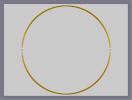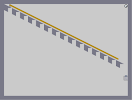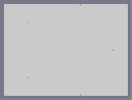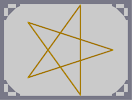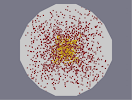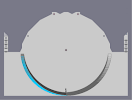Vernalic-Lateral Decay Linar Death Star Lateral Equinox EXperimneto Blue Gene

Pages: (0)

### ...

so all you did was invert x and y axis...and change the dimensions to fit...great...why is this here again?

did i miss something??

### Nice

I never was on the verge of doing that....5/5!
Keep it up!

### HOW BORED???

how bored were you to do that?????

### Well,

clicking that box would mean that I didn't put any input into making the map. But I wrote the script so it really isn't necessary. Also, one could tell just by the map that my program would have to be required for it. Thanks anyways krusch.

### This is really sweet...

just like all of the others 5/5. And thanks a bunch for the ded.!!! Keep up the fantastic work!

### You should..

..tick that box which says that the map was made with a mapmaking tool.
But make sure you say you made the program yourself, unless you didn't.
: /